The goal of this chapter is to start applying the laws of hydrodynamics that were provided in Eqs. (7.32) and (7.42) to problems of interest in acoustics. By applying these laws to some simple acoustical networks, we can begin to develop our understanding of their meaning and their broad utility. We start by ignoring dissipationFootnote 1 and by choosing acoustical elements that are small compared to the wavelength of sound. In this nondissipative lumped-element approximation, the continuity equation (7.32) leads us to the definition of an acoustical compliance, C, that plays the same role as a capacitor in alternating current (AC) electrical circuit theory or a spring in the theory of mechanical vibrations.

Under those same approximations, the Euler equation (7.42) leads to the definition of an acoustical inertance, L, which is equivalent to an inductor in the electrical analogy, or a mass in the theory of mechanical vibrations. If a capacitor and inductor (or spring and mass) are combined, an electrically (mechanically) resonant (tuned) circuit is created. In acoustics, the combination of an acoustical inertance and acoustical compliance is called a Helmholtz resonator. If you have ever blown over the neck of a beverage bottle and produced a tone, you have excited a Helmholtz resonator. If you haven’t, now’s the time! (Fig. 8.1)

The other important electrical circuit element, not mentioned above, is the electrical resistor, Rdc. In an electrical circuit, the capacitor can store electrostatic potential energy, EC = (½) CV2, and the inductor can store magnetic potential energy, EL = (½) LI2, but neither of those idealized circuit elements can dissipate energy; that is the role of the resistor, 〈Πelt = (½)RdcI2. Our acoustical elements, the inertance and compliance, are also idealized and do not dissipate energy because neither the Euler equation (7.42) nor the continuity equation (7.32) contains terms that are dissipative.

We will introduce dissipation from the viscous flow losses in the inertance that arise from the $$\mu {\nabla}^2\overrightarrow{v}$$ term in the Navier-Stokes equation (7.34). The dissipation introduced by the compliance arises from the fact that the pressure oscillations of the gas contained within the compliance produce temperature changes in the gas (7.25). Since the solid walls of the compliance (volume) have a much larger heat capacity than the gas within, they remain nearly isothermal. The gas far from the walls is adiabatic, so there will be irreversible entropy generation, $${\dot{s}}_{gen}>0$$, as shown in Eq. (7.46), produced by the $$\overrightarrow{\nabla}\cdotp \kappa\;\overrightarrow{\nabla}T$$ term in the entropy equation (7.43) due to the heat flowing back and forth between the gas and the walls of the container. The thermal and viscous dissipation will provide our acoustically resistive lumped elements, playing a role analogous to the electrical resistance, Rdc, or the mechanical resistance, Rm. The origin of their dissipative behavior and the resulting consequences will be examined in the Chap. 9.

Thus far, we have used harmonic analysis extensively in Part I. It is the acoustician’s most powerful analytical tool. For vibrating systems, whether discrete masses and springs or distributed continua, like strings, bars, membranes, and plates, the equilibrium condition usually corresponded to the relevant coordinate being set equal to zero (e.g., the equilibrium position of a mass at xo = 0 or the displacement of the undisturbed string, y (x, t) = 0). Acousticians express the temporal and spatial response of oscillating fluid parameters (i.e., pressure, density, velocity, temperature, and entropy) as the sum of a mean (equilibrium) value and a harmonic deviation (i.e., sinusoidally varying in space and/or time) of that parameter away from its mean value. In that way, acousticians can effortlessly convert sets of coupled differential and integral equations into a much simpler set of coupled algebraic equations.

Let us take as our first example the pressure of a fluid in which there is an acoustic disturbance from equilibrium that varies both in three-dimensional space, $$\overrightarrow{x}$$, and in time, t.

$$p\left(\overrightarrow{x},t\right)={p}_m\left(\overrightarrow{x}\right)+{p}_1\left(\overrightarrow{x},t\right)$$
(8.1)

The mean pressure, pm ($$\overrightarrow{x}$$), is a constant in time but may vary slowly over space.Footnote 2 Most commonly in acoustics, the variation in pm ($$\overrightarrow{x}$$) in space might be due to changes in depth for ocean acoustics or due to changes in altitude for propagation of sound in the atmosphere. We will examine both those possibilities later, but in most instances, pm ($$\overrightarrow{x}$$) will be assumed constant in both space and time.

The deviation from the equilibrium pressure in Eq. (8.1) is represented as p1(x, t). The meaning of the subscript m is obvious from its specification as the “mean” value. The subscript “1” on p1 indicates that it is the first-order (linear) deviation from equilibrium. This linear deviation from equilibrium is sometimes called the instantaneous value, because it is constantly changing, typically with sinusoidal time dependence. The subscripting choice is insignificant as long as you do not have to consider nonlinear effects. The velocity amplitude, v1, is a linear deviation from equilibrium, so the kinetic energy density, $$\left({KE}_2\right)/V=\left(\frac{1}{2}\right){\rho}_m{v}_1^2$$, is quadratic, where now the subscript “2” indicates that the kinetic energy density is a second-order quantity in its deviation from equilibrium. If there is no mean flow, there are no first-order terms in the kinetic energy.

All of the relevant acoustic variables in our single-component fluid can be expanded in the same way as we expanded the pressure in Eq. (8.1). The acoustic approximation assumes that the deviation from equilibrium is much smaller than the equilibrium value: |p1| ≪ pm. That was certainly true for the case of the loud (115 dBSPL) sound wave used as an example at the start of Chap. 7, where |p1|/pm = 1.6 × 10−4 (160 ppm). Just as we did for the pressure, all of the other acoustic variables can be expanded in a similar way:

$$\rho \left(\overrightarrow{x},t\right)={\rho}_m\left(\overrightarrow{x}\right)+{\rho}_1\left(\overrightarrow{x},t\right)$$
(8.2)
$$T\left(\overrightarrow{x},t\right)={T}_m\left(\overrightarrow{x}\right)+{T}_1\left(\overrightarrow{x},t\right)$$
(8.3)
$$s\left(\overrightarrow{x},t\right)={s}_m\left(\overrightarrow{x}\right)+{s}_1\left(\overrightarrow{x},t\right)$$
(8.4)

As before, the acoustic approximation requires that |ρ1| ≪ ρm, |T1| ≪ Tm and |s1| ≪ sm. In fact, for an adiabatic process, s1 ≡ 0.

The velocity is treated in a slightly different way. For most of the cases of interest to us, $${\overrightarrow{v}}_m=0$$. Instead, for the first-order particle velocity, $${\overrightarrow{v}}_1$$, the acoustic approximation requires that it be small compared to the sound speedFootnote 3, c.

$${M}_{ac}=\frac{\left|{\overrightarrow{v}}_1\right|}{c}<<1$$
(8.5)

Mac is a dimensionless variable called the acoustic Mach number. When individual components of the velocity are needed, we will write $${\overrightarrow{v}}_1=u{\hat{e}}_x+v{\hat{e}}_y+w{\hat{e}}_z$$, as will be done in Eq. (8.10).

In most cases, an acoustician is concerned with the first-order deviation of a parameter from its equilibrium value. Furthermore, this first-order deviation is assumed to be a sinusoidal function of space and time. The assumption of harmonic behavior is not as restrictive as it may seem. Fourier’s theorem (see Sect. 1.4) guarantees that any continuous, periodic (though possibly non-sinusoidal) function can be represented as a sum of sinusoidal functions if the system exhibits linear behavior.

These definitions allow the acoustically induced deviations from equilibrium to be expressed as complex exponentials that provide a particularly convenient functional form for integration or differentiation, as we saw in Part I. For example, we can express the deviation of the pressure from its equilibrium value for a wave traveling to the left or to the right as we did for waves on strings in Chap. 3.

$${p}_1\left(\overrightarrow{x},t\right)=\mathit{\Re e}\left[\hat{\mathbf{p}}{e}^{j\left(\omega\;t\mp \overrightarrow{k}\cdotp \overrightarrow{x}\right)}\right]$$
(8.6)

As before, the minus sign (−) in the exponential corresponds to propagation in the $$+\overrightarrow{x}$$ direction, and the plus sign (+) corresponds to propagation in the $$-\overrightarrow{x}$$ direction. The complex pressure amplitude, $$\hat{\mathbf{p}}$$, is a phasor that combines both amplitude and phase. By using complex notation, differentiation with respect to time corresponds to a simple multiplication of p1(x, t) by +jω. Differentiation with respect to position corresponds to a simple multiplication of p1(x, t) by $$\pm j\overrightarrow{k}$$, with the choice of sign depending on whether the wave is moving in the +x direction (−jk) or in the –x direction (+jk).

For the one-dimensional standing wave, like those first treated on strings in Sect. 3.3.1, the spatial dependence can be characterized by a trigonometric function and the time dependence by a complex exponential. The form below assumes that the standing wave has a pressure anti-node located at x = 0.

$${p}_1\left(\overrightarrow{x},t\right)=\mathit{\Re e}\left[\hat{\mathbf{p}}{e}^{j\omega\;t}\right]\cos (kx)$$
(8.7)

With this discussion and the assumed behavior of first-order deviations from equilibrium shown in Eqs. (8.6) and (8.7), the restriction of “small compared to a wavelength,” where λ = 2π/k = c/f, should be clear for both standing and traveling waves. We are now prepared to derive expressions for acoustical compliance and acoustical inertance from the hydrodynamic equations.

## 2 Acoustical Compliance and the Continuity Equation

Acoustics is a branch of fluid dynamics. Our initial approach will be to study the motion of a fluid particle in response to the forces applied to it by adjacent fluid particles. Before we can start that study, we need to decide on our frame of reference. In fact, that choice has already been made by the form in which we chose to express the equations of hydrodynamics in Eqs. (7.32), (7.34), and (7.43). Implicit in the form of those equations was the decision to choose a laboratory frame of reference also known as an Eulerian coordinate system.

In the study of fluids, there are two choices. One is the choice typically made in classical mechanics, where we write equations that describe the time evolution of each particle’s coordinates in space and time. Since our system is typically composed of Avogadro’s number of particles, we define a fluid particle (or fluid parcel) as a volume of fluid that contains enough atoms or molecules (billions) so that the fluid in the volume can be treated as a continuous medium, yet small enough so that all acoustic variables are nearly constant throughout that small volume. In effect, we are imaging that we could color those billion atoms or molecules that constitute our “particle” red and then follow the time evolution of that red spot. That choice is designated the Lagrangian description.

A Lagrangian description might be convenient if you are using “tracer particles” (e.g., smoke in air) and have a laser Doppler vibrometer that follows the motion of those tracer particles optically. For most laboratory measurements, it is simpler to assume that your sensors (e.g., microphone, hydrophone, thermocouple, hot-wire anemometer, etc.) are at a fixed location (in the laboratory frame of reference) and the fluid is moving past the sensor. We will be using that Eulerian description almost exclusively in this textbook.

The simplification provided by the Eulerian frame of reference, which is useful when we interpret the signals from our sensors, comes at the cost of having to redefine “acceleration.” The acceleration must include both the “local” time rate of change of a variable within our fluid parcel at the location of interest, plus the change in the variable caused by transport (convection) of the value of that variable into and out of our location of interest from some neighboring position. The term in square brackets below and in Eqs. (7.34), (7.42), and (7.43) is called (total) convective derivative.

$$\frac{D}{Dt}\equiv \left[\frac{\partial }{\partial t}+\left(\overrightarrow{v}\cdotp \overrightarrow{\nabla}\right)\right]$$
(8.8)

The convective component of the acceleration in Eulerian coordinates that arises from applying the convective derivative to the fluid velocity, $$\overrightarrow{v}\left(\overrightarrow{x},t\right)$$, will be explained in Sect. 8.4.1, where the Euler equation is exploited to derive the acoustical inertance.

### 2.1 The Continuity Equation

What happens to a fluid parcel when exposed to a temporally and spatially varying pressure, a sound wave, for example? Two things happen: (i) the mass of the fluid within the parcel changes (the density of the fluid changes), and (ii) the velocity of the fluid within the parcel changes. The continuity equation, which is also known as the conservation of mass equation, expresses that density change in terms of the variation in mass flux, $$\rho \overrightarrow{v}$$, as a function of position.

$$\frac{\partial \rho }{\partial t}+\nabla \cdotp \kern0.5em \left(\rho \overrightarrow{v}\right)=0$$
(8.9)

We can examine the consequence of those changes by examining the Eulerian volume shown in Fig. 8.2. The rate of mass flowing into the volume from the left is mass flux, $$\left(\rho \overrightarrow{v}\right)$$, times the differential area, dA = dy dx, evaluated at the left side of the differential volume. The rate of mass flow out of the volume on the right side is the same product evaluated at the right side. The rate of increase of mass inside the volume, $$\partial m/\partial t=\dot{m}$$, is the difference in the mass flow rate in and the mass flow rate out. The fact that Eq. (8.9) is a homogeneous equation guarantees that there are no sources or sinks of mass within the differential Eulerian volume, dV.

The form of the continuity equation can be justified by first considering this one-dimensional flow, diagrammed in Fig. 8.2, with the mass flow restricted to occurring only the x direction. To simplify our mathematical expressions (by elimination of subscripts), we will let the Cartesian components of the vector velocity be u, v, and w, so that $$\overrightarrow{v}=u{\hat{e}}_x+v{\hat{e}}_y+w{\hat{e}}_z$$. If the flow is only in the x direction, then v = w = 0. We will also let u be only a function of x: u = u(x).

The net change of mass, dm, during a time interval, dt, within the differential volume, dV, due to a fluid with mass density, ρ, moving with a velocity, u, in the x direction, can be written as the difference between the x component of the mass flux at x, (ρu)x, times the differential area, dA = dy dz, times the time interval, dt, minus the mass that flows out from the same area located at x + dx.

$$dm={\left(\rho u\right)}_x dy\; dz\; dt-{\left(\rho u\right)}_{x+ dx} dy\; dz\; dt$$
(8.10)

Expanding (ρu)x + dx in a Taylor series about x and retaining only the first (linear) term in the series provide an expression for (ρu)x + dx in terms of (ρu)x and the partial derivative of (ρu) with respect to x, evaluated at x.

$$\begin{array}{c}{\left(\rho u\right)}_{x+ dx}={\left(\rho u\right)}_x+{\left(\frac{\partial \left(\rho u\right)}{\partial x}\right)}_x dx\kern0.5em \\ {}\Rightarrow {\left(\rho u\right)}_x-{\left(\rho u\right)}_{x+ dx}=-{\left(\frac{\partial \left(\rho u\right)}{\partial x}\right)}_x dx\kern1em \end{array}}$$
(8.11)

This allows us to determine the time rate of change of the mass, $$\dot{m}=\partial m/\partial t$$, and hence, the change in fluid density, ρ, within that differential Eulerian volume, dV.

$$\dot{m}=\frac{dm}{dt}=\frac{\partial \rho }{\partial t} dx\kern0.35em dy\kern0.35em dz=-{\left(\frac{\partial \left(\rho u\right)}{\partial x}\right)}_x dx\kern0.35em dy\kern0.35em dz\kern1em \Rightarrow \kern1em \frac{\partial \rho }{\partial t}+\frac{\partial \left(\rho u\right)}{\partial x}=0$$
(8.12)

The right-hand version of Eq. (8.12) is the continuity equation in one dimension, since we have assumed that there is flow only in the x direction. Expanding to three dimensions, we recover the full equation of continuity that was first introduced in Eq. (7.32) without justification.

$$\frac{\partial \rho }{\partial t}+\nabla \cdotp \kern0.5em \left(\rho \overrightarrow{v}\right)=0$$
(8.13)

In the above derivation, u, v, and w were used as the x, y, and z components of the velocity vector so that we did not end up with double subscripts (e.g., (ρvx)x). Elsewhere, $$\overrightarrow{v}$$ has been used as the velocity vector with Cartesian components vx, vy, and vz. The coexistence of these two velocity component designations (as well as their equivalents in cylindrical or spherical coordinates) should not cause any confusion.

### 2.2 Linearized Continuity Equation

Starting with the simplest case, we now consider the small length of pipe shown schematically in Fig. 8.3, filled with a compressible fluid at pressure, p. By “small,” we mean that Δx ≪ λ and A1/2 ≪ λ; both the diameter and the length of the element are much less than the wavelength of sound at the frequencies of interest.

Since we (as acousticians) are “timid hydrodynamicists,” and since Fig. 8.3 represents one-dimensional flow along the x axis, we will “linearize” the continuity equation in Eq. (8.12) before we apply it to the lumped-element representation (acoustical compliance) of the small section of tube in Fig. 8.3. We substitute the expansion of the fluid mass density about equilibrium from Eq. (8.2) into the one-dimensional version of the continuity equation (8.12), and we will linearize the resulting expression by discarding any second-order terms that include products of two first-order deviations from equilibrium. Subsequently, we will demonstrate (quantitatively) that the elimination of the quadratic term is justified if u ≪ c.

$$\frac{\partial \left({\rho}_m+{\rho}_1\right)}{\partial t}+\frac{\partial }{\partial x}\left[\left({\rho}_m+{\rho}_1\right)u\right]=0$$
(8.14)

Recognizing that ρm is a constant, so (∂ρm/∂t) = 0, the square bracket in Eq. (8.14) can be expanded.

$$\frac{\partial {\rho}_1}{\partial t}+\frac{\partial }{\partial x}\left[{\rho}_mu+{\rho}_1u\right]=0$$
(8.15)

The constant coefficient, ρm, can be taken outside the spatial derivative.

$$\frac{\partial {\rho}_1}{\partial t}+{\rho}_m\frac{\partial u}{\partial x}+\frac{\partial \left({\rho}_1u\right)}{\partial x}=0$$
(8.16)

At this point, we recognize that the (ρ1u) term is a quadratic (second-order) combination of two first-order deviations from the equilibrium state. For that reason, this term can be eliminated from Eq. (8.16) to produce the linearized one-dimensional version of the continuity equation.

$$\frac{\partial {\rho}_1}{\partial t}+{\rho}_m\frac{\partial u}{\partial x}=0$$
(8.17)

We can determine the limits on the validity of excluding the (ρ1u) term by using harmonic analysis to convert the differential equation (8.17) into an algebraic equation by assuming that ρ1 and u are represented by a right-going traveling wave with c = ω/k.

$$j\omega \hat{\boldsymbol{\uprho}}-{\rho}_m jk\hat{\mathbf{u}}=0\kern1em \Rightarrow \kern1em \frac{\hat{\boldsymbol{\uprho}}}{\rho_m}=\frac{\hat{\mathbf{u}}}{c}\kern1em \Rightarrow \kern1em \frac{\left|\hat{\mathbf{u}}\right|}{c}\equiv {M}_{ac}<<1$$
(8.18)

We can take the ratio of the term we discarded in Eq. (8.16) to the term we kept to produce Eq. (8.17), again assuming a right-going traveling wave.

$$\frac{\frac{\partial \left({\rho}_1u\right)}{\partial x}}{\rho_m\frac{\partial u}{\partial x}}=\frac{- jk\hat{\boldsymbol{\uprho}}\hat{\mathbf{u}}}{-{\rho}_m jk\hat{\mathbf{u}}}=\frac{\hat{\boldsymbol{\uprho}}}{\rho_m}\kern1em \Rightarrow \kern1em \frac{\left|\hat{\boldsymbol{\uprho}}\right|}{\rho_m}={M}_{ac}<<1$$
(8.19)

As long as we are in the low-amplitude acoustic limit (Mac ≪ 1), then the quadratic term in Eq. (8.16) is negligible compared to the two terms which survived in our linearized continuity equation (8.17).

Before continuing to examine the consequences of the linearized continuity equation, it is worthwhile to make a temporary digression and reflect on what effects we might have been eliminating by rejecting the second-order (ρ1u) term in Eq. (8.16).

The time average of any harmonic first-order acoustic variable over an integer number of periods, nT, will be identically zero. That is not true for the time average, 1u>t, of that quadratic term.

$${\left\langle {\rho}_mu\right\rangle}_t=\frac{\rho_m}{T}\underset{0}{\overset{T}{\int }}u\; dt=0\kern1em \mathrm{but}\kern1em {\left\langle \left({\rho}_m+{\rho}_1\right)u\right\rangle}_t=\frac{1}{T}\underset{0}{\overset{T}{\int }}\left({\rho}_m+{\rho}_1\right)\;u\; dt\ne 0$$
(8.20)

Both ρ1 and u vary sinusoidally with time, so their product will be proportional to sin(ω t) sin (ω t + ϕ) = (½)[1 +  cos (2ω t)] cos ϕ + (½) sin (2ω t) sin ϕ. The time average of the two trigonometric terms that contain (2ωt) in their arguments will vanish, but the time average of the constant is non-zero. This corresponds to a second-order mass flux, $${\dot{m}}_2$$, that is driven by the product of the two first-order variables.

The non-zero time-averaged flow can be understood by recognizing that the density variation, ρ1, and the acoustic flow velocity, u, are in-phase for a one-dimensional traveling wave. During the first half-cycle, ρ = ρm + ρ1(t), which is greater than the mean value, ρm, while u is positive, corresponding to mass flow toward the right. That net mass flow transports a second-order mass flux of $${\dot{m}}_2(right)$$ to the right during the first half-cycle. During the second half-cycle, ρ(t) is smaller than the mean value, ρm, while u is negative, transporting a mass flux, $${\dot{m}}_2(left)$$, to the left. Those two mass flows are not equal and result in a net flow of mass to the right: $${\dot{m}}_2={\dot{m}}_2(right)-{\dot{m}}_2(left)>0$$ . This nonlinear effect is known as streaming, and the second-order heat flux carried by this acoustically driven flow can be detrimental to the operation of heat engines and refrigerators .

We are not yet ready to apply our linearized continuity equation (8.17) to the small element in Fig. 8.3 because the fluid variable in that figure is p, not ρ. How do we address this problem? We invoke the equation of state that relates pressure and density: ρ = ρ (p, T). For this application, let us assume that the fluid which fills the tube in Fig. 8.3 is an ideal gas and that the compressions and expansions of that gas occur adiabatically, so using Eq. (7.20),  = const. The adiabatic gas law can be differentiated logarithmically (see Sect. 1.1.3) to obtain an expression that relates pressure variations, δp, to density variations, δρ.

$$\ln p-\gamma \ln \rho =\ln \left[\mathrm{constant}\right]\kern1em \Rightarrow \kern1em \frac{\delta p}{p_m}=\frac{p_1}{p_m}=\gamma \frac{\delta \rho}{\rho_m}=\gamma \frac{\rho_1}{\rho_m}$$
(8.21)

Since we used the adiabatic gas law to create Eq. (8.21), we can use the ratio of δp to δρ that provides the conversion between density variations and pressure variations.

$$\frac{\delta p}{\delta \rho}={\left(\frac{\partial p}{\partial \rho}\right)}_s=\frac{p_1}{\rho_1}=\frac{\gamma {p}_m}{\rho_m}=\frac{\gamma \mathit{\Re T}}{M}\equiv {c}^2$$
(8.22)

The Ideal Gas Law of Eq. (7.4) has been used to convert the ratio of pm/ρm to ℜT/M, where ℜ is the universal gas constant, T the absolute [kelvin] temperature, and M the atomic or molecular mass [kg/mol] of the ideal gas.

As will be shown later, c2 is the square of the adiabatic sound speed in an ideal gas. Substituting ρ1 = ρm (p1/γpm) from Eq. (8.22) into the linearized continuity equation (8.17), we obtain a version of the one-dimensional, linearized continuity equation that is now only valid for an ideal gas under adiabatic conditions, but that contains p1 as the thermodynamic variable instead of ρ1.

$$\left(\frac{1}{\gamma {p}_m}\right)\frac{\partial {p}_1\left(x,t\right)}{\partial t}+\frac{\partial u\left(x,t\right)}{\partial x}=0$$
(8.23)

Having defined a volume velocity, U1 = uA, with units of [m3/s], that is the product of the fluid velocity, u, and the cross-sectional area of the element, A, Eq. (8.23) can be re-written in terms of the volume velocity. Since Δx ≪ λ, the second term in Eq. (8.23) can be approximated as −ΔU1/AΔx = −ΔU1/V, where V is the volume of the lumped element represented in Fig. 8.3. Finally, converting to complex notation for a single-frequency wave,

$$\frac{j\omega}{\gamma {p}_m}\hat{\mathbf{p}}-\frac{\Delta \hat{\mathbf{U}}}{V}=0$$
(8.24)

In fluid dynamics, it is also common to define a mass flow rate, $$\dot{m}={\rho}_m{U}_1$$, that has units of [kg/s]. The concept of volume velocity is so useful in acoustics, particularly for analysis of sound sources (e.g., loudspeakers), that acousticians generally prefer U1 to $$\dot{m}$$.

### 2.3 Acoustical Compliance

The expression for the one-dimensional, linearized, single-frequency continuity equation, as written in Eq. (8.24), will allow us to define an acoustical compliance for the short tubular element, shown in Fig. 8.3, that would be analogous to the capacitance for an electrical capacitor or the reciprocal of stiffness for a mechanical spring. By introducing the concept of a complex acoustical impedance, $${\mathbf{Z}}_{\mathbf{ac}}\equiv \hat{\mathbf{p}}/\hat{\mathbf{U}}$$, we can make such an analogy.Footnote 4

$${\mathbf{Z}}_{\mathbf{ac}}\equiv \frac{\hat{\mathbf{p}}}{\hat{\mathbf{U}}}=\frac{1}{j\omega}\frac{\gamma {p}_m}{V}$$
(8.25)

Again, we recognize that ΔU = U1, which is the deviation of the volume velocity from its mean value, usually taken to be zero, Um = 0.

The expression for the capacitive reactance of a capacitor is XC = 1/(ωC). If we compare that expression to Eq. (8.25), the definition of an acoustical compliance, C, follows directly by analogy.

$$C=\frac{V}{\gamma {p}_m}=\frac{V}{\rho_m{c}^2}$$
(8.26)

The right-hand version simply invokes the result of Eq. (8.22), where c2 is the adiabatic sound speed in an ideal gas.

Just like the capacitive impedance, the acoustical impedance of this acoustic compliance is given as Zac = 1/jωC. The analogy is represented schematically using electrical component symbols in Fig. 8.4.

### 2.4 The Gas Spring

We can revisit the idealized cylinder from Fig. 7.2, which is closed at one end and has a leak-tight frictionless piston at the other, and then examine the concept of an acoustic compliance from another perspective by forcing the piston to undergo harmonic displacements, $$x(t)=\mathit{\Re e}\left[\hat{\mathbf{x}}{e}^{j\omega\;t}\right]$$, as shown in Fig. 8.5.

Logarithmic differentiation of the adiabatic equation of state for an ideal gas from Eq. (7.19) provides a relationship between the change in volume, dV(t) = Ax(t), and the change in pressure, p1(t).

$${pV}^{\gamma }=\mathrm{constant}\kern1em \Rightarrow \kern1em \frac{p_1(t)}{p_m}=-\gamma \frac{dV(t)}{V}=-\gamma \frac{A}{V}x(t)$$
(8.27)

If we assume that the mean pressure, pm, on both sides of the piston is the same, then (8.27) can be re-written in the form of Hooke’s law: F =  − Κx.

$$F=-{\mathrm{K}}_{gas}{x}_1={p}_1A=-\gamma {p}_m\frac{A^2}{V}{x}_1\kern1em \Rightarrow \kern1em {\mathrm{K}}_{gas}=\frac{\gamma {p}_m}{V}{A}^2={\rho}_m{c}^2\frac{A^2}{V}$$
(8.28)

This corresponds to a spring constant, Κgas, for the “gas spring” that was usedFootnote 5 in Chap. 7, Problem 4.

Using harmonic analysis, the piston’s complex (phasor) volume velocity amplitude, $$\hat{\mathbf{U}}$$, can be related to the time derivative of its position, $$\hat{\mathbf{x}}:\hat{\mathbf{U}}= j\omega\;\hat{\mathbf{x}}A$$. Substitution of x1 into the differential form of the Adiabatic Gas Law in Eq. (8.27) reproduces Eq. (8.24) that was used to define the acoustical compliance, C, in Eq. (8.26), if we assume that the fluid that enters the compliance due to the non-zero volume velocity, U, shown in Figs. 8.3 and 8.4, cannot leave the cylinder, or equivalently, U = ΔU.

## 3 Hydrostatic Pressure

Here come those Santa Ana winds again.” Steely Dan 

Before we calculate the acoustic inertance of a small element using the linearized Euler equation, as we just did to calculate an acoustic compliance using the linearized continuity equation, we will make a particularly simple application of the Navier-Stokes equation (7.34) to calculate the variation of pressure with depth for a stagnant fluid in a gravitational field characterized by a gravitational acceleration, $$\overrightarrow{g}$$. Since we are assuming a stagnant fluid, all terms in (7.34) that contain velocity will vanish.

$$\overrightarrow{\nabla}p=\rho \overrightarrow{g}\kern1em \mathrm{if}\kern1em \overrightarrow{v}=0$$
(8.29)

Applying this to water, which we will assume is incompressible, means that the density will not be a function of depth, ρ ≠ ρ(z). If the direction of the gravitational acceleration, $$\overrightarrow{g}$$, is downward in the z direction, only the z component of p changes with depth. The integration of Eq. (8.29), to provide the pressure as a function of depth, is then straightforward.

$$\frac{dp}{dz}=\rho g\kern1em \Rightarrow \kern1em {\int}_0^{-z} dp=\rho g{\int}_0^{-z} dz\kern1em \Rightarrow \kern1em p(z)={p}_o-\rho g z\kern0.5em \mathrm{with}\kern0.5em z\le 0$$
(8.30)

If we take our fluid to be an ocean or a lake, then the constant of integration, po, is just the atmospheric pressure at the surface. The hydrostatic pressure increases linearly with depth if the water is assumed to be incompressible. If we take the density of water to be 103 kg/m3, then dp/dz ≅ 104Pa/m ≅ 1 bar/10 m.

The same calculation can be done easily (though a bit unrealistically) for an isothermal atmosphere. In that case, the density of air will depend upon altitude. The pressure and density are related by the Ideal Gas Law (7.4), so ρm(p) = pm(M/ℜT). Since the height of the Earth’s atmosphere is generally taken to be less than 100 km and the mean radius of the Earth is R = 6378 km, the variation in g (z) should be less than 0.8%. Assuming that g ≠ g (z) and letting the altitude increase in the +z direction from sea level, Eq. (8.29) can also be integrated.

$${\int}_0^h\frac{dp}{p}=\ln \left[\frac{p}{p_o}\right]=\frac{gM}{\mathit{\Re T}}h\kern1em \Rightarrow \kern1em {p}_m(h)={p}_o{e}^{-h/\mu }$$
(8.31)

The scale length, μ ≡ ℜT/gM, can be evaluated under standard atmospheric conditions , at sea level with T = 288.15 K (15 °C), where g (0) = 9.8066 m/s2 and M = 28.9644 kg/kmole, resulting in a characteristic “exponential atmospheric thickness” of μ = 8435 m. The standard value of atmospheric pressure at sea level is po = 101,325 Pa and ρo = 1.2250 kg/m3 at 15 °C. Note that the acceleration due to gravity at that altitude, g (μ) = 9.7807 m/s2 . This deviates from its value at sea level, g (0), by less than ¼ %. The pressure (and density) of an isothermal atmosphere therefore decreases exponentially with increasing altitude. Equation (8.31) can be evaluated to determine the initial decrease in atmospheric pressure from sea level with height under isothermal conditions: (∂p/∂h)h = 0 = −po/μ ≅ −12 Pa/m.

If instead we let the density of the atmosphere be constant, so p(h) = po − ρogh, then Eq. (7.13) can be used to determine the adiabatic rate of change of temperature with increasing height.

$$dQ={c}_P dT- dp/\rho =0\kern1em \Rightarrow \kern1em {c}_P dT=- gdz\kern1em \Rightarrow \kern1em {\left(-\frac{\partial T}{\partial h}\right)}_s=\frac{g}{c_P}\equiv {\Gamma}_d$$
(8.32)

Γd is known as the dry (hence, the d subscript) atmospheric lapse rate. For dry air at 15 °C, cP = 1005 J/kg-°C, so Γd ≅ −9.8 °C/km. This is more realistic than the previous isothermal assumption because weather is usually transporting large parcels of air up and down, changing their pressure and temperature adiabatically. In reality, neither T nor p is independent of height in the troposphere, and the lapse rate is less than Γd because of the latent heat of water .

For any resident of the Los Angeles basin, the atmospheric conditions that bring hot air westward from the Mojave and Sonoran deserts that then are heated adiabatically as they descend into the basin are known as “Santa Ana winds,”Footnote 6 hence the quote that started this section.

## 4 Inertance and the Linearized Euler Equation

Just as we did with the calculation of the compliance of a small lumped element in Sect. 8.2.3, we will begin our calculation of the inertance of a small lumped element by linearizing the relevant hydrodynamic equation. In this case, we ignore viscous and gravitational forces in Eq. (7.34) and begin with the Euler equation (7.42), reproduced below.

$$\frac{D\overrightarrow{v}}{Dt}=\frac{\partial \overrightarrow{v}}{\partial t}+\left(\overrightarrow{v}\cdotp \overrightarrow{\nabla}\right)\overrightarrow{v}=-\frac{\overrightarrow{\nabla}p}{\rho }$$
(8.33)

### 4.1 The Venturi Tube

Some students find the convective contribution to the acceleration term, $$\left(\overrightarrow{v}\bullet \overrightarrow{\nabla}\right)\overrightarrow{v}$$, in the total derivative, $$D\overrightarrow{v}/ Dt$$, of Eq. (8.8) and the Euler equation (8.33), to be a bit mysterious. This mystery might be cleared up if we look at a situation where ∂v/∂t = 0 everywhere in the fluid, but the fluid’s acceleration is non-zero. The Venturi tube flow meter,Footnote 7 shown schematically in Fig. 8.6, is a simple case that will be easy to analyze .

If we assume the drawing in Fig. 8.6 depicts a rectangular duct of constant width but the linearly varying cross-section between x = 0 and x = L, reducing the cross-sectional area by a factor of two, which is filled with an incompressible fluid, (∂ρ/∂t = 0), then conservation of mass (7.32) guarantees that the velocity, $$\overrightarrow{v}$$, must increase linearly within the constriction between x = 0 and x = L.

$$\overrightarrow{v}(x)={v}_{left}\left(1+\frac{x}{L}\right){\hat{e}}_x\kern1em \mathrm{for}\kern1em 0\ge x\ge L$$
(8.34)

As a function of time, $$\partial \overrightarrow{v}(t)/\partial t=0$$, but since the velocity depends upon position, the convective acceleration is non-zero.

$$\left(\overrightarrow{v}\cdotp \overrightarrow{\nabla}\right)\overrightarrow{v}={v}_x\frac{\partial {v}_x}{\partial x}{\hat{e}}_x+{v}_y\frac{\partial {v}_y}{\partial y}{\hat{e}}_y+{v}_z\frac{\partial {v}_z}{\partial z}{\hat{e}}_z$$
(8.35)

Since $$\overrightarrow{v}$$ along the center line is only a function of x, only the first term on the right-hand side of Eq. (8.35) is non-zero. This convective acceleration can be related to the pressure gradient, $$\overrightarrow{\nabla}p\cong \left({p}_{left}-{p}_{right}\right)/L$$, by substitution into the Euler equation (8.33).

$$\rho \left({v}_x\frac{\partial {v}_x}{\partial x}\right)=-\frac{\partial p}{\partial x}$$
(8.36)

The above version can be integrated to produce the pressure difference, Δp = pleftpright, that would lead to a height difference, Δh = Δp/ρg, in the two standpipes shown at x < 0 and x > L in Fig. 8.6.

$$-{\int}_{left}^{right} dp=\rho {\int}_{left}^{right}{v}_x\;{dv}_x\kern1em \Rightarrow \kern1em \Delta p=\frac{\rho }{2}\left({v}_{right}^2-{v}_{left}^2\right)$$
(8.37)

This result is a manifestation of the Bernoulli equation that is very important in nonlinear acoustics for understanding radiation pressure  and acoustical levitation . For our purposes here, it illustrates that there can be accelerations and pressure gradients in fluids where $$\partial \overrightarrow{v}(t)/\partial t=0$$ everywhere within the fluid.

### 4.2 The Linearized Euler Equation

The convective term in the acceleration is manifestly second order (in the absence of steady flow) since it is proportional to the product of two first-order quantities, $${\overrightarrow{v}}_1\cdotp \kern0.5em {\overrightarrow{v}}_1$$. We can use the same technique of harmonic analysis as used previously in Eq. (8.19), this time to determine the conditions that justify neglect of the convective acceleration term, using sound speed, c = ω/k.

$$\left|\frac{\left(\overrightarrow{v}\cdotp \overrightarrow{\nabla}\right)\overrightarrow{v}}{\partial \overrightarrow{v}/\partial t}\right|=\left|\frac{j k{\hat{\mathbf{v}}}^2}{j\omega \hat{\mathbf{v}}}\right|=\frac{\left|\hat{\mathbf{v}}\right|}{c}\kern1em \Rightarrow \kern1em \frac{\left|\hat{\mathbf{v}}\right|}{c}={M}_{ac}<<1$$
(8.38)

We can neglect the second-order term in Eq. (8.33),$$\left(\overrightarrow{v}\cdotp \overrightarrow{\nabla}\right)\overrightarrow{v}$$, compared to the first-order term, $$\partial \overrightarrow{v}/\partial t$$, if the velocity amplitudes are within the acoustic approximation: Mac ≪ 1.

As before, when we eject a particular term from the fundamental governing equations, it takes along with it many interesting phenomena. In this case, the loss of the convective acceleration term, $$\left(\overrightarrow{v}\cdotp \overrightarrow{\nabla}\right)\overrightarrow{v}$$, removes our ability to understand the formation of shock waves and sonic booms. It also eliminates any non-zero time-averaged acoustic forces that are related to the Bernoulli pressure, 〈p2t = (ρm/2)v2 . These forces include radiation pressure , the levitation of solid objects in intense standing wave fields,Footnote 8 and the manipulation of such levitated objects to cause rotations (acoustic torques) and vibrations .

To linearize the part of the Euler equation that remains after removal of the convective acceleration, the density can be expanded into its mean value, ρm, plus the first-order variation, ρ1, as shown in Eq. (8.2).

$$\frac{\partial {\overrightarrow{v}}_1}{\partial t}=-\frac{\overrightarrow{\nabla}{p}_1}{\rho_m\left(1+{\rho}_1/{\rho}_m\right)}$$
(8.39)

The acoustic approximation guarantees that ρ1m = Mac ≪ 1, so it can also be removed from Eqs. (8.33) and (8.39) to produce the linearized Euler equation that we will use to derive an expression for the lumped inertance of the small fluid element with all dimensions much less than the wavelength of sound.

$$\frac{\partial {\overrightarrow{v}}_1}{\partial t}=-\frac{\overrightarrow{\nabla}{p}_1}{\rho_m}$$
(8.40)

Before doing so, we can interpret Eq. (8.40) again using the Eulerian fluid particle shown in Fig. 8.7.

The force exerted on the left side of the differential volume, dV, is p(x)dy dz. The force exerted on the right side is p(x + dx)dy dz. The net force, Fnet, is their difference.

$${F}_{net}=\left[p(x)-p\left(x+ dx\right)\right]\kern0.5em dy\kern0.5em dz$$
(8.41)

p (x + dx) can be expanded in a Taylor series about x, as indicated by the subscript on the partial derivative.

$$p\left(x+ dx\right)=p(x)+\left(\frac{\partial p}{\partial x}\right) dx$$
(8.42)

Using this result, the net force on the Eulerian volume element can be expressed in terms of the pressure gradient in the x-direction: ∇x p = (∂p/∂x).

$${F}_{net}=\left[p(x)-p\left(x+ dx\right)\right]\kern0.5em dy\; dz=-\left(\frac{\partial p}{\partial x}\right) dx\; dy\; dz=-\left(\frac{\partial p}{\partial x}\right) dV$$
(8.43)

Newton’s Second Law of Motion states that this net force must be equal to the mass of fluid contained within dV times its acceleration. Since we have assumed a pressure gradient only in the x direction, we will again express the vector velocity in Cartesian components:

$$\left(\rho\;dV\right)\frac{\partial u}{\partial t}=-\left(\frac{\partial p}{\partial x}\right) dV\kern1em \Rightarrow \kern1em \rho \frac{\partial u}{\partial t}=-\left(\frac{\partial p}{\partial x}\right)$$
(8.44)

This is just the one-dimensional version of the linearized Euler equation that was derived in Eq. (8.40) from the hydrodynamic equation (8.33).

### 4.3 Acoustical Inertance

Consider a short pipe of constant cross-sectional area, A, filled with an incompressible fluid. For oscillations at a single frequency, we again let $$\hat{\mathbf{U}}$$ be the complex amplitude of the oscillatory volume velocity through the element as shown in Fig. 8.8. We can apply the one-dimensional linearized Euler equation (8.44) to the element, assume time-harmonic variables, and make a finite-difference approximation to the one-dimensional pressure gradient, $${\nabla}_xp\cong -\Delta \mathit{\Re e}\left[\hat{\mathbf{p}}{e}^{j\omega\;t}\right]/\Delta x$$.

$$j\omega \frac{\hat{\mathbf{U}}}{A}=\frac{1}{\rho_m}\frac{\Delta \hat{\mathbf{p}}}{\Delta x}$$
(8.45)

The inertance, L, can be obtained by rearranging Eq. (8.45) to take the form of an acoustical impedance, Zac, as was done previously for acoustical compliance, C, in Eq. (8.25).

$${\mathbf{Z}}_{\mathbf{ac}}\equiv \frac{\Delta \hat{\mathbf{p}}}{\hat{\mathbf{U}}}=j{\omega \rho}_m\frac{\Delta x}{A}$$
(8.46)

As with our derivation of the acoustical compliance, the acoustical inertance can be identified by analogy to the inductive reactance of AC circuit theory: XL = ωL.

$$L=\frac{\rho_m\Delta x}{A}$$
(8.47)

Just as with the compliance, C, the inertance, L, can be represented by an electrical circuit element (inductor) as shown in Fig. 8.9.

### 4.4 Acoustical Mass

As with the equivalent gas stiffness, Κgas, of an acoustical compliance expressed in Eq. (8.28), we can interpret our expression for acoustical inertance in Eq. (8.47) as the inertial mass of incompressible fluid, oscillating within the inertance element, due to the time-harmonic pressure gradient across it. We use Newton’s Second Law of Motion since it represents the dynamical equivalent of the fluid-mechanical Euler equation: F = ma. The mass of fluid, m, contained within the short inertance element is the incompressible fluid’s density times the volume, m = ρmx A), and its acceleration amplitude is $$\hat{\mathbf{a}}=\left( j\omega \hat{\mathbf{U}}/A\right)$$.

$$\begin{array}{c}\hat{\mathbf{F}}=\Delta \hat{\mathbf{p}}A=\left({\rho}_m\Delta xA\right)\frac{j\omega \hat{\mathbf{U}}}{A}\\ {}\Rightarrow \frac{\hat{\mathbf{p}}}{\hat{\mathbf{U}}}=\frac{\left(\hat{\mathbf{F}}/A\right)}{\hat{\mathbf{U}}}\equiv j\omega L=\left({\rho}_m\Delta xA\right)\frac{j\omega}{A^2}\kern1em \Rightarrow \kern1em L=\frac{\rho_m\Delta x}{A}\end{array}}$$
(8.48)

At this point, it could be legitimate to complain that we were able to obtain expressions for the acoustical inertance, L, and the acoustical compliance, C, of a “lumped element” directly from Newton’s Second Law and the Adiabatic Gas Law. Why were we forced to spend so much time working through the hydrodynamic equations? Of course, the answer is that the goal of the hydrodynamic derivations of both L and C was to familiarize you with those equations, as well as to define parameters that will be useful in acoustical network analyses, since they will be applied throughout this textbook to a variety of acoustical problems.

Nondissipative, linearized lumped-element analysis was only our first and our simplest application of hydrodynamics. Their utility will now be demonstrated in the analysis of Helmholtz resonators, which are important in a variety of applications (e.g., noise control, musical acoustics, and optimization of loudspeaker enclosure performance).

## 5 The Helmholtz Resonance Frequency

Having derived expressions (in two different ways!) for the acoustical inertance and acoustical compliance of elements whose dimensions are all small compared to the wavelength of sound, we are now in a position to join those “lumped elements” together to form an acoustical network. To do so, we need to know the joining conditions. What are the quantities that are continuous at the interface between any two elements?

In this case, the answer will be simple because the variables, p and U, were chosen in our definitions of the acoustical inertance of Eq. (8.47) and the acoustical compliance of Eq. (8.26), specifically to make it easy to join the elements of differing cross-sectional areas to each other. We know that p is continuous across an interface, both because of Pascal’s law and because the linearized Euler equation (8.40) would lead to infinite accelerations if there were non-zero pressure differences across the interfacial boundary of infinitesimal thickness.

The continuity equation requires that fluid cannot accumulate at the interface between elements (which has no volume), therefore the mass flux must also be continuous. As before, the mass flux and the volume velocity are intimately related, $$\dot{m}=\rho U$$, so an equivalent requirement is that the volume velocity also be continuous across the interface if the density is continuous, which usually implies that the temperature is the same on both sides of the interface (Fig. 8.10).

Our first application of this “lumped-element” model will be to an acoustical network that has both historical and contemporary significance: the Helmholtz resonator. It was introduced by H. L. F. Helmholtz (1821–1894) in his classic book On the Sensations of Tone, published in 1862, in Chapter III, titled “Analysis of Musical Tones by Sympathetic Resonance.”

If we examine Fig. 8.11, it is clear that continuity of volume velocity, U, is quite different from continuity of particle velocity, v. To conserve mass, the velocity of fluid flow in the neck of the Helmholtz resonator, vneck, will be much larger than the velocities of the fluid near the neck either inside the volume, vvolume, of the resonator or in front of the neck. The streamlines for the flow transition will be bent , but if the amplitude of the oscillation is sufficiently small, the transition will be smooth.Footnote 9 At higher amplitudes, there could be all kinds of turbulent effects, such as vortex shedding and jetting, which will be irreversible and thus dissipative. These effects can be very important in high-amplitude resonators, but for the present linearized analyses, we are restricting our attention to acoustical networks that oscillate by an infinitesimal amount away from their equilibrium state. At this point in our investigations, if such high-amplitude effects occur, then we would just simply decrease the excitation amplitude until they disappear.

In the days before the advent of electroacoustic transducers and amplifiers that could convert sound waves into electrical signals whose frequency content could be determined by electronic filters, spectrum analyzers, and FFT signal analyzers, acousticians could use Helmholtz resonators placed in their ears to determine the frequency of sounds. (Another option was to compare the observed tone to the frequencies of a set of tuning forks, like those shown in Fig. 5.11). Figure 8.12 shows a set of Helmholtz resonators that were used in an acoustic laboratory near the end of the nineteenth century.

As long as 12,000 years ago, Helmholtz resonators were used as musical instruments (e.g., ocarinas) in Asia and Mesoamerica . Another early use of the Helmholtz resonator was as whistles, one version of such a whistle is shown in Fig. 8.13. Peruvian whistling bottles were made in pre-Columbian Peru from 500 BCE (Salinar and Gallinazo cultures along Peru’s North Coast) until the Spanish conquest of the Incas (1150 AD) . Today, Helmholtz resonators are used extensively as sound absorbers in architectural applications and as tuned “sound traps” in recording studios, ducts, and engine mufflers. A hollow brick that is used as a resonant absorber in rooms is shown in Fig. 8.14 .

### 5.1 Helmholtz Resonator Network Analysis

We can analyze the response of our Helmholtz resonator by drawing the equivalent circuit shown schematically in Fig. 8.15. For this analysis, we will assume that the resonator is placed in an externally generated sound field with pressure amplitude, $$\left|\hat{\mathbf{p}}\right|$$, and with angular frequency, ω, so that outside the neck of the resonator, $$p(t)=\mathit{\Re e}\left[\hat{\mathbf{p}}{e}^{j\omega\;t}\right]$$. Since the end of the compliance that is not joined to the neck is sealed, that pressure can only be applied to the compliance through the neck (inertance). For that reason, the “high” end of the voltage generator, representing the externally imposed pressure, is connected to the one end of the neck but is “grounded” to the closed end of the compliance (compare Fig. 8.11 with Fig. 8.15).

The volume velocity that is able to enter the compliance through the resonator’s neck represents the resonator’s response to the oscillating pressure imposed beyond the neck. Before calculating the amplitude and phase of the (complex) amplitude of the volume velocity as a function of the frequency, $$\hat{\mathbf{U}}\left(\omega \right)$$, it is useful to consider the limits of the response at frequencies well above and below the resonance frequency of the resonator, ωo, where the impedance of the inertance, ZL = jωL, and the impedance of the compliance, ZC = 1/jωC, exactly cancel each other.

From DC to frequencies less than ωo, the gas flows easily through the neck into the compliance, and the volume velocity amplitude, $$\hat{\mathbf{U}}$$, is controlled by the compliance, in accordance with the definition of compliance in Eq. (8.26): $$\hat{\mathbf{U}}\left(\omega <{\omega}_o\right)\cong j\omega\;C\;\hat{\mathbf{p}}$$. In this case, because the reactance of the inertance, XL = ωL, is much smaller than that of the reactance of the compliance, XC = (ωC)−1, the amplitude of the pressure difference between the ends of the neck, $$\Delta \hat{\mathbf{p}}$$ (see Figs. 8.11 and 8.15), is negligible for small ω and $$\left|\hat{\mathbf{p}}\right|\ge \left|{\hat{\mathbf{p}}}_{cav}\right|$$.

At frequencies above resonance, ωo, the inertance of the neck controls how much gas can flow into the compliance: $$\hat{\mathbf{U}}\left(\omega >{\omega}_o\right)\cong \hat{\mathbf{p}}/\left( j\omega\;L\right)$$. At sufficiently high frequencies, the neck blocks flow into the compliance so $$\left|{\hat{\mathbf{p}}}_{cav}\left(\omega \gg {\omega}_o\right)\right|\ll \left|\hat{\mathbf{p}}\right|$$, as long as the frequency is still well below that for excitation of the first standing wave mode of the neck, ω1, shown on the right-hand side of Fig. 8.14, or the resonance frequencies of the volume (see Chap. 13 and particularly Sect. 13.4 if the volume is spherical).

With those frequency limits in mind, we can write down the general expression for the amplitude of the volume velocity that enters the compliance through the neck: $$\hat{\mathbf{U}}\left(\omega \right)=\hat{\mathbf{p}}/\mathbf{Z}\left(\omega \right)$$. With L and C “in series,” the total acoustical impedance, Z(ω), is just the sum of the neck’s acoustical impedance, jωL, and the volume’s acoustical impedance, jωC.

$$\mathbf{Z}\left(\omega \right)={\mathbf{Z}}_{\mathbf{L}}+{\mathbf{Z}}_{\mathbf{C}}= j\omega L+\frac{1}{j\omega\;C}$$
(8.49)

The acoustical impedance, as written in Eq. (8.25), determines the amplitude of the oscillating component of the pressure, $${\hat{\mathbf{p}}}_{cav}$$, inside the compliance, based on the control of the amplitude of the volume velocity, $$\hat{\mathbf{U}}\left(\omega \right)$$, that is imposed by the acoustical impedance in Eq. (8.49).

$${\hat{\mathbf{p}}}_{cav}=\frac{\hat{\mathbf{U}}}{j\omega\;C}=\frac{1}{j\omega\;C}\left[\frac{\hat{\mathbf{p}}}{j\omega L+{\left( j\omega\;C\right)}^{-1}}\right]$$
(8.50)

The term within the square brackets in Eq. (8.50) incorporates the substitution $$\hat{\mathbf{U}}\left(\omega \right)=\hat{\mathbf{p}}/\mathbf{Z}\left(\omega \right)$$. When the denominator within the square brackets vanishes, the theory (in its current dissipationless form!) predicts that the pressure inside the compliance will become infinite, since Z (ωo) = 0 at the Helmholtz resonance frequency.

$${\omega}_o=\frac{1}{\sqrt{LC}}=c\sqrt{\frac{A}{V\Delta {x}_{neck}}}$$
(8.51)

The divergence of $$\left|{\hat{\mathbf{p}}}_{\mathbf{cav}}\right|$$ as ω approaches ωo will be eliminated when we add dissipation to our lumped-element model using DeltaEC in Sects. 8.6.7 and 8.6.8 and in the analyses provided in Sect. 9.4.4 that apply the thermoviscous boundary layer losses. The acoustic pressure amplitude in the compliance is “amplified” by the resonance near ωo and attenuated far above ωo.

$$\frac{{\hat{\mathbf{p}}}_{cav}}{\hat{\mathbf{p}}}\cong \frac{{\mathbf{Z}}_{\mathbf{C}}}{{\mathbf{Z}}_{\mathbf{C}}+{\mathbf{Z}}_{\mathbf{L}}}=\frac{\omega_o^2}{\omega_o^2-{\omega}^2}\kern1em \mathrm{if}\kern1em \omega \ne {\omega}_o$$
(8.52)

It is also important to consider the phase of $${\hat{\mathbf{p}}}_{cav}/\hat{\mathbf{p}}$$ at low and at high frequencies compared to ωo, based on the right-hand term in Eq. (8.50). At ω ≪ ωo, fluid easily enters the compliance, and both p (t) and pcav (t) are in-phase; when the pressure outside the volume is high, the pressure inside the volume is high also. For ω ≫ ωo, the sign in the denominator in Eq. (8.50) becomes negative indicating that p (t) and pcav (t) are 180 degrees (π radians) out-of-phase. That phase reversal is exploited to invert the phase of the radiation from the rear of a loudspeaker cone in a bass-reflex loudspeaker enclosure so it will add to the pressure produced by the front of the loudspeaker, as discussed in Sect. 8.7. In that case, the Helmholtz resonator is used to enhance the low-frequency output of a loudspeaker.

### 5.2 A 500-mL Boiling Flask

With Eq. (8.51), we are now in a position to calculate the frequency of a Helmholtz resonator and compare measured results to the theory. Substitution of the resonator dimension and sound speed, provided in the caption of Fig. 8.16, into Eq. (8.51) predicts fo = ωo/2π = 245.3 Hz. The experimentally determined frequency is fexp = 213.8 Hz. The measured frequency is nearly 13% lower than the calculated result. That discrepancy is far greater than expected based on our ability to accurately determine the flask’s physical dimensions or our ability to measure the resonance frequency (about ±1.5 Hz out of 214 Hz or approximately ±0.7%).

As we will see later, this discrepancy is mostly a result of the fact that the oscillating gas flow does not stop abruptly neither at the lip of the neck nor at the neck’s entrance into the spherical volume of the boiling flask.Footnote 10 The gradual transition of the flow from the oscillations within the neck to the stagnant gas surrounding the exterior of the resonator and inside of the volume is usually represented by a “radiation mass,” which we will calculate when we study the radiation from a baffled piston (e.g., loudspeaker) in Sect. 12.8.3 and an unbaffled piston in Sect. 12.9. That additional mass increases the inertance of the neck and is usually incorporated into Eq. (8.51) by defining an “effective length,” Δxeff > Δxneck, that is the sum of the physical length (in this case, Δxneck = 49.2 mm) plus some constant times the radius of the neck.Footnote 11

Although we are not ready to make a theoretical calculation of an effective length correction for the neck in our example, we can use Eq. (8.51), and the measured frequency, to make an experimental determination of the neck’s effective length based on the measured Helmholtz resonance frequency, fexp.

$$\Delta {x}_{eff}={\left(\frac{c}{2\pi {f}_{\mathrm{exp}}}\right)}^2\frac{A}{V}$$
(8.53)

Substitution of the measured value for ΔV = 0 in Fig. 8.17 into Eq. (8.53) produces a value of Δxeff = 64.7 mm, corresponding to a length correction of Δxeff  Δxneck = 15.5 mm = 1.24a, where a = Dneck/2 is the radius of the neck.

In principle, the effective length is independent of the volume of the Helmholtz resonator, as long as the flow contours representing the streamlines from the neck are not perturbed by interactions with the closed end of the volume. We can test this assumption by changing the volume, V, of the resonator. This can be accomplished by injecting measured quantities of water (assumed to be incompressible) into the boiling flask using the apparatus in Fig. 8.16. Neither the sound speed, c, the neck area, A, nor the neck length, Δxneck, would be affected by these incremental changes in volume, ΔVi.

Equation (8.51) can be rearranged to allow the square of the measured period, $${T}_i^2={f}_i^{-2}$$, to be plotted against the added volume of water, ΔVi.

$$\left[\frac{c^2}{4{\pi}^2}A\right]{T}_i^2=\Delta {x}_{eff}\left({V}_o-\Delta {V}_i\right)$$
(8.54)

The slope of the best-fit line in Fig. 8.17 will determine Δxeff. The empty volume of the resonator, Vo, will also be determined by the fit: Vo = −(intercept)/(slope).

It is visually apparent from the quality of the least-squares straight-line fit to the data in Fig. 8.17 that Eq. (8.51) and Eq. (8.54) do an excellent job of representing the behavior of the Helmholtz resonator. The relative uncertainty in the slope can be related to the square of the correlation coefficient for the fit, R2 = 0.9989 (see Sect. 1.9.2), producing a relative uncertainty in the effective length of ±1.5%. That is a little better than what would be predicted based on a relative uncertainty in the resonant frequency measurement (±0.7%) and an estimate of the relative uncertainty in the volume changes of about 1 ml ÷ 50 ml = 2%. It is also encouraging that the ratio of the intercept to the slope produced an effective empty volume for the resonator of 502.4 ml.

## 6 DeltaEC Software

To this point, we have not yet exploited the power of digital computation beyond the generic spreadsheet and curve-fitting programs provided by mass-marketed commercial software packages. Several software packages have been developed specifically to serve the acoustic community. In underwater acoustics, ray-tracing programs are very popular, as are commercial packages such as LMS Sysnoise  used for modeling sound in three-dimensional spaces, BassBox Pro  for designing loudspeaker enclosures, or X-OverPro for designing loudspeaker crossovers.

The program that has become an important tool for thermoacousticians  since 1985 is the Design Environment for Low-amplitude Thermoacoustic Energy Conversion (DeltaEC). Drs. G. W. Swift and W. W. Ward developed it at Los Alamos National Laboratory for design and analysis of thermoacoustic engines, refrigerators, and gas mixture separators. It was updated in 2013 to provide an improved user interface with the help of John Clark . Although it has the capability to model heat exchangers and porous media used in such thermoacoustic systems, DeltaEC is also well-suited to the design and/or analysis of any quasi-one-dimensional acoustical network consisting of ducts, horns, waveguides, compliances, branches, flow impedances, and loudspeakers in a variety of fluid media bounded by a variety of solids.Footnote 12

Its results have been tested extensively in laboratories worldwide, and it executes calculations very quickly. This high computational speed is accomplished by using analytical results for the transverse variation in the complex pressure and velocity fields. For example, in a CONE segment, solutions to the Webster horn equation  provide the pressure as a function of position even through the cross-sectional area is changing and there are non-zero radial components of the fluid’s velocity.

DeltaEC numerically integrates along one spatial dimension using a low-amplitude, acoustic approximationFootnote 13 assuming sinusoidal time dependence, ejωt. It simultaneously integrates the continuity equation (7.32), the Navier-Stokes equation (7.34), and other equations, such as the energy equation, in a geometry specified by the user’s choice of a sequence of “segments,” such as ducts, compliances, transducers, heat exchangers, and thermoacoustic stacks or Stirling regenerators. DeltaEC always assumes steady-state conditions and harmonic time dependence of all acoustic variables.

We will use DeltaEC initially to revisit the 500 ml flask used as a Helmholtz resonator in the example of Sect. 8.5.2. Since DeltaEC includes the dissipative effects that we have ignored thus far, it will provide some additional performance information, as well as serve as a familiarization exercise for the software.

The DeltaEC software installer is also available at the Springer web site for this textbook and the Springer site for Swift’s textbook Thermoacoustics: A Unifying Perspective for Some Engines and Refrigerators. .

You will have to unzip (Mac) or execute (Windows) the file. That process also installs a copy of the DeltaEC User’s Guide. The manual is in ∗.pdf format and contains searchable hyperlinked text. We will only be concerned with the first four chapters of the Guide, but the Reference Sections (Chaps. 8, 9, 10, 11 and 12) are very useful once you have learned the basics. The Reference Sections also contain the equations that are implemented in the software.

This textbook will employ a “two-pronged approach” to familiarize you with some of the elementary acoustical functions that are provided by DeltaEC. The primary sources of information are the first four chapters of the User’s Guide. The first chapter provides some background on the software and how it functions. The second chapter introduces the user interface by modeling the 1992 Penn State Championship Bottle both as a Helmholtz resonator and as an open-closed standing wave resonator of variable cross-section (see Problem 6 at the end of this chapter). The plotting features of DeltaEC are covered in the third chapter, and the reverse Polish notation (RPN) segment, which lets the user perform customized calculations within a model, is covered in the fourth chapter and introduced here (briefly) toward the end of Sect. 8.6.8.

Although the DeltaEC User’s Guide should be your primary reference for exploitation of DeltaEC software, the Guide will be augmented in this textbook by providing several examples in the remainder of this section and the next. These examples are directly related to acoustical networks that have already been analyzed and will apply DeltaEC to model a standing wave resonator, the 500 ml Helmholtz resonator from Sect. 8.5.1, three coupled Helmholtz resonators in Sect. 8.7, and a bass-reflex loudspeaker enclosure based on the Helmholtz resonator model in Sect. 8.8.

### 6.2 Getting Started with DeltaEC (Thermophysical Properties)

After installing the DeltaEC software on all of your computers, a DeltaEC icon should appear on each computer’s desktop near the lower left corner of the screen. You can open DeltaEC by double-clicking on the icon. The design of that icon is motivated by the thermal core of a traveling-wave thermoacoustic engine developed at Los Alamos National Laboratory .

DeltaEC usually requires the thermophysical properties of both the fluid supporting the wave and the properties of the solid that contains the fluid in each segment to execute the integrations. We can access this feature directly to have DeltaEC provide these data. When you open DeltaEC by clicking on the icon (instead of opening an existing ∗.out file), you will be presented with a “window” that is blank but has pull-down menus at the top. Under the “Help” menu, you can access the User’s Guide. Under the “Tools” menu, you can access the ThemoPhys(ical) Prop(erties) window shown in Fig. 8.18. That window can also be opened from the keyboard by typing “t” when the computer’s attention is on the main DeltaEC window.

As our first example, we’ll use the environmental conditions under which the algebraic results for the 500 ml Helmholtz resonator (Figs. 8.16 and 8.17) were calculated to provide the equilibrium fluid parameters for the air inside that resonator. In the Thermophysical Properties window, modify the gas type to be “air” using the pull-down arrow, the frequency to be 245.3 Hz, and the pressure to be standard atmospheric pressure of 101,325 Pa. Since DeltaEC variables are specified exclusively in MKS units, the temperature must be input in absolute [kelvin] units. The data for our 500 ml example was taken at 22.5 °C, so input the absolute temperature, Tm = (22.5 + 273.15) K = 295.65 K, into the “Temp(K)” window.

With all of those values appearing in their appropriate location, use your mouse to click on “Show.” This should instantly generate the requested thermophysical properties of air under the specified conditions as shown in Fig. 8.19.

The thermophysical property file header in Fig. 8.19 includes the fluid type (air), the mean temperature (Tm = 295.65 K), and the mean pressure (pm = 101,325 Pa). The second line contains the frequency-independent fluid properties and their associated units. The first four parameters in the top line are the ratio of specific heats “gamma,” sound speed “a(m/s),”Footnote 14 density “rho(kg/m^3),” and specific heat at constant pressure “cp (J/kg/K).” These parameters should already be familiar.

The subsequent transport parameters will be discussed in Chap. 9, where the dissipative terms in Eqs. (7.34) and (7.43) are analyzed. The isobaric coefficient of thermal expansion, βp = − (1/ρ) (∂ρ/∂T)p, “beta (1/K),” is just the reciprocal of the absolute temperature, Tm, for an ideal gas. In our case, βp = (1/Tm) = 0.33824 × 10−2 K−1, agreeing with the DeltaEC output to the five decimal places displayed. Next in line are the thermal conductivity, “k(W/m/K)”; the dimensionless Prandtl number (see Sect. 9.5.4), “Prandtl”; and the shear viscosity, “mu(kg/s/m).”

The bottom data line in Fig. 8.19 lists the frequency, “frequency=,” and two frequency-dependent results: the viscous boundary layer thickness, “delta_nu=,” also known as the viscous penetration depth, δν, and the thermal boundary layer thickness, “delta_kappa=,” aka the thermal penetration depth, δκ, which will be derived and discussed thoroughly in Chap. 9.

### 6.3 Creating planewave.out

There are several ways to create a DeltaEC model of an acoustical network. The result of such a model is a ∗.out file that consists of a sequence of segments like those shown in Fig. 8.21. One can start “from scratch,” but I generally prefer to modify an existing file using the file editing commands in DeltaEC.

We will start by creating a file “from scratch” that represents a simple plane wave resonator of constant cross-section that is shown schematically in Fig. 8.22 and will result in the DeltaEC model shown in Figs. 8.20 and 8.21. Once we gain some experience with the way DeltaEC expects you to insert segments and input the parameters contained within each segment, we will modify planewave.out to create a DeltaEC model of the Helmholtz resonator example based on the 500 mL boiling flask in Sect. 8.5.2. To begin creating “planewave.out,” you should have already downloaded DeltaEC from the Los Alamos Lab’s “Thermoacoustics Home Page.”

If DeltaEC is still open (because you were working on the thermophysical example), you can go to the “File” pull-down menu tab, and click “New.” That action will produce a file with a single BEGIN segment and title the model “NewModel.” Next to “NewModel” is “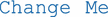” in a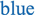font. By double-clicking on an item in afont, you are able to change it. Double-click on “Change Me” and type in “Planewave Resonator.” There will also be a box that will let you add comments, but we won’t put in any comments, so just click the “OK” button.

Before going further, now would be a good time to save the file. This is done by going to the “File” drop-down menu and selecting “Save As.” Chose the directory in your computer where you would like to save this file (a convenient choice is the same folder that holds the DeltaEC program), and then title the file “planewave.out.” You will see that the “tab” at the top of the DeltaEC model will change its name from “NewModel” to “planewave.” As we add segments, it is a good idea to “Save” the file at regular intervals or before quitting.

BEGIN Segment. All DeltaEC models start with a BEGIN segment that specifies the “global” features of the model, like the gas or liquid type (e.g., helium, air, humid air, sodium, etc.); the mean fluid pressure, pm; the mean temperature of the fluid at the start of the model, Tm; and the acoustic frequency, f. You can see these parameters when you click on the “+” sign at the left of BEGIN. This will “unpack” the segment and let you see the parameters it specifies. For very long models, being able to unpack individual segments with the “+” or “compact” with the “−” can be convenient.

In the unpacked form, you see seven parameters infont that we are free to modify. For this example, we’ll accept the default values for (0a) = Mean P = 100 kPa, (0b) = Freq = 100 Hz, and the beginning temperature, (0c) = TBeg = 300 K. We’ll also accept the default Gas Type as. We can ignore the “Optional Parameters” for now. The use of the segment number and parameter in parentheses is a convenient way to identify parameters in the model. Notice again that all parameters are specified in MKS units.

Our intention will be to drive this resonator with a sinusoidally varying volume velocity. We’ll set the initial volume velocity magnitude, (0f) = |U| = 0.010 m3/s, by clicking on the blue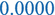in the (0f) position. Doing so should bring up the “Parameter Edit: 0f |U|” window. Type 0.01 into the “Value” line and then click “OK.” That should assign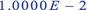m3/s as the value for (0f). By leaving (0 g) = Ph (U) = 0, we define all other phases in the model with respect to the phase of the driving volume velocity.

Since we are specifying the volume velocity that will drive the resonator, we will rely on DeltaEC to calculate the resulting acoustic pressure magnitude, |p|, and the phase of that pressure, Ph (p), with respect to Ph (U) = 0 deg. at any frequency, Freq = (0b). To impose this choice, we can double-click on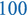that is the default value in (0d) to open the “Parameter Edit: 0d |p|” window. Type in 1000 for the Value, check the “Set as Guess” box, and click “OK.” This brings up a Gues label to the left of (0d) to remind us that DeltaEC will be allowed to modify this value of $$\left|\hat{\mathbf{p}}\right|$$ to satisfy the model and its boundary conditions. It also puts our guess for pressure in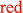font to warn us that its value is not based on a solution of the model: (0d) = |p| =Pa. Since we don’t know the phase of the pressure with respect to volume velocity, we also need to make (0e) = Ph (p) a guess. Just click on the default valueto bring up the “Parameter Edit: 0e Ph(p),” check the “Set as Guess” box, and click “OK.”

This indicates that the values are only “guesses” and DeltaEC’s “shooting method” has been given “permission” to change those values to satisfy constraints placed on the model by the quantities that have been designated “targets.” In this file, those “targets” will be the rigid (infinite) impedances specified in the final HARDEND segment, (4a) and (4b). That completes the BEGIN segment.

SURFACE Segment. Now we can specify the area of the “piston” that will produce the volume velocity we specified in (0f). Although we could just let the BEGIN segment produce that volume velocity, since the end of the resonator has some surface area, including a SURFACE segment after the BEGIN segment will mean that any dissipation on that “end cap,” acting as a piston, will be included in our model. To add this next segment, just “right click” in the model, and an “Append” option will appear with a list of possible segments listed alphabetically. You may have to use the arrow to scroll down to see SURFACE. When it appears, just click on it, and DeltaEC will add the new SURFACE segment to your model.

It is useful to name the segment, so click on “” that is to the right of SURFACE, type in “Piston Face,” and then click “OK.” Again, we will not include any comments. SURFACE has only one parameter and we’ll set it to (1a) = Area = 0.01 m2. Just click onto bring up the “Parameter Edit: 1a Area” window, enter a “Value” of 0.01, and then click “OK.” SURFACE is a solid, so the segment allows specification of “Solid Type.” Accept the default value of “.” “Ideal” just means that we want DeltaEC to assume the solid has infinite heat capacity and infinite thermal conductivity, resulting in a surface that holds the gas isothermal at the gas-solid interface.Footnote 15 If you double-click on “,” it will bring up the menu of other built-in solid materials (e.g., stainless steel, copper, mylar, etc.). All physical segments in DeltaEC require specification of the gas type and/or solid type.

Unlike the BEGIN segment, the SURFACE segment has a list of six “Results,” from (0A) = |p| to (0F) = Edot, at the right-hand side of the segment. All of the numerical results are infont to warn us that the model has not run and that the current values are only placeholders. After the program has run successfully, all of the results that are currently shown inwill change to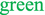, indicating the upgrade in the model status to actual “results.”

The results will be discussed once the model is complete and has run successfully in Sect. 8.6.4. Now might be a good time to “Save” the file to include the newest segment either by using the “File” drop-down menu or by simply clicking on the “floppy disk” icon on the banner beneath the drop-down menus.

DUCT Segment. We now add the resonator, a tube of circular cross-section. “Right click” in the model to activate the “Append” option and choose DUCT. Click on “” that is to the right of DUCT, name this segment “Resonator Body,” and then click “OK.” To specify the DUCT’s cross-sectional area (2a), click on the default value to bring up the “Parameter Edit: 2a Area” window. This time we will not enter a value but instead click on the “SameAs” button. Since the only other parameter in our model with units of area is (1a), DeltaEC has guessed that (1a) might be a good choice. This is correct, so just click “OK.” That will make the area of the DUCT the same as the area of the SURFACE (piston) segment. Choosing to link those areas makes it easy to change the cross-sectional area of the entire model by just changing the cross-sectional area of the piston.

DeltaEC has also chosen to make a “Master-Slave” linkage. It automatically chose the DUCT’s Perimeter (2b) to be equal to 0.35449 m. DeltaEC guessed that our DUCT was circular and made the Perimeter, $$\Pi =\sqrt{4\pi A}$$. It also placed a notice to the right of (2a) and (2b) to remind us of that link with “Mstr 2a” to indicate that the value of (2a) is controlling the value of (2b). Again, if we chose to modify the cross-sectional area of the DUCT, then that Master-Slave link would keep the DUCT’s cross-section circular. To accommodate ducts of different shape, the perimeter of the duct (2b) can be specified independently from its area (2a). You can see that choice if you double-click on Master-Slave Links to bring up the dialog box that has made the default choice: “Maintain constant perimeter as area changes.” Double-click on the Length (2c) and make the length of the DUCT be 5.00 m.

Although DeltaEC claims to work for “low-amplitude” acoustics, there are features that allow models to incorporate some nonlinear fluid dynamics to accommodate higher amplitudes. Double-click on theto open a dialog box that will let you choose low-amplitude (laminar) flow or high-amplitude (turbulent) flow. Click on the “Laminar” button. For turbulent flow, you would also have to specify a surface roughness factor.

SURFACE Segment. Append another SURFACE segment to represent the other end of the resonator. Name that segment “End Cap,” then double-click on the Area, and make it “SameAs” (2a).

HARDEND Segment. The final HARDEND segment is a “logical” segment that is required to complete the file. It is one of only two possible choices, the other being SOFTEND. Use “Append” to add the final HARDEND segment and rename it as “Rigid Termination.”

In a SOFTEND segment, the real and imaginary parts of the impedance are specified by the user. If the SOFTEND is infinitely “soft,” then both the real part of the impedance, R (4a), and the imaginary part, I (4b), are zero. Of course, there are other choices that would make sense in other situations. For example, the real part of the SOFTEND impedance could be made equal to ρmc/A for a duct to create an anechoic termination that would make the solution a traveling wave instead of a standing wave, as discussed in Sect. 3.6.3 for a string with a resistive termination.

For an ideal HARDEND, the impedance is infinite. Since ∞ is a difficult concept for a computer, HARDEND requires specification of the real and imaginary parts of the complex admittance, which is the (complex) reciprocal of the impedance. For an infinitely rigid and lossless HARDEND condition, ℜe [1/z= ℑm [1/z] =, as shown in lines (4a) and (4b) of Fig. 8.21.

We will make each of those rigid boundary conditions a “Target” that DeltaEC will attempt to satisfy. This is done by double-clicking on “.” That will bring up a dialog box that allows us to select the first two of three possible targets. Check the box next to (4a) to make the real (i.e., dissipative) part of the admittance a target, R(1/z) = 0, and check the second box (4b) to make the imaginary (i.e., reactive) part of the admittance, I(1/z) = 0, also a target. To the left of both of those entries is “Targ.” If you double-click on zero in either (4a) or (4b), you will open the dialog box that shows that the “Set Target” box has been checked. This now designates the values specified in (4a) and (4b) as “targets” that DeltaEC’s “shooting method” will try to make the results in 4G and 4H equal to those targets by modifying the values of guesses, 0d and 0e.

At this point, you should be sure to “Save” the model and then compare it to the screenshot in Fig. 8.21. If you click the “/” icon in the banner, the entire model should collapse to just the segment titles as shown in the screenshot in Fig. 8.20. Click on that icon again and all of the segments for the entire model should “unpack.”

Figure 8.22 provides a schematic diagram of the physical resonator’s parts that are modeled in the planewave.out file shown above the “schematic” generated by DeltaEC’s “View Schematic” function available under the “Display” pull-down menu. I like to check the schematic view since it is drawn to scale. If I’ve entered a geometrical parameter incorrectly (e.g., a length in centimeters instead of meters), then the schematic view will look incorrect.

### 6.4 Running planewave.out

There are two ways to run DeltaEC. One way is to just let the program integrate its way through a file starting at the BEGIN statement. If complex $$\hat{\mathbf{p}}$$ and $$\hat{\mathbf{U}}$$ are specified in the BEGIN segment, then DeltaEC just integrates its way through the model, matching the complex values (both magnitude and phase) of $$\hat{\mathbf{p}}$$ and $$\hat{\mathbf{U}}$$ at the interfaces between segments of the model. I find that I rarely use that mode because most models have constraints that are not specified in the BEGIN segment and those constraints determine the values of $$\hat{\mathbf{p}}$$ and $$\hat{\mathbf{U}}$$ in all of the segments, including BEGIN, as was the case with the HARDEND condition in segment (#4) that dictated the complex pressure in the BEGIN segment (#0), given the amplitude of the excitation by the assumed volume velocity of magnitude, |U| = 0.01 m3/s, in (0f).

Most of the time, I use the “equation solver” mode that lets DeltaEC adjust the “guesses” in an attempt to achieve the “targeted” values. The “shooting method” requires that there be an equal number of guesses and targets.Footnote 16 They can be viewed at any time by going to the “Display” pull-down menu and selecting “Guesses Targets.” You can also access this display from the keyboard by typing “g” while the computer’s focus is on the main DeltaEC window.

The particular choice of targets and guesses shown in Fig. 8.23 implies that we will be asking DeltaEC to determine the response of the modeled system to a volume velocity of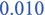m3/s imposed on one end of the resonator at a frequency ofHz.

The time has come to run planewave.out. The run starts either (i) by clicking on therun arrowhead at the top of the DeltaEC window, (ii) by going to the “Tools” pull-down menu and selecting run, or (iii) by typing “r.” DeltaEC responds by generating a “Run Monitor” window that is shown in Fig. 8.24. We can view the Guesses Targets window again and see how the guesses have changed. We see that under the specified conditions (|U| =m3/sec and Freq. =Hz), the pressure at the piston location (0d) is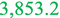Pa and the phase difference between $$\hat{\mathbf{U}}$$ and $$\hat{\mathbf{p}}$$ at that location is°.

Looking back at the expanded planewave.out file, we see that all of the results in the right-hand column and both guesses, (0d) and (0e), have changed fromtoindicating that the solver has found a self-consistent solution.

### 6.5 Finding the Resonance Frequencies of planewave.out

As we might imagine, there is nothing special about operation at 100 Hz. We could ask DeltaEC’s solver to find the fundamental half-wavelength, λ/2, resonance frequency, f1. Before doing so, let’s run Thermophysical Properties to get the speed of sound under the current conditions within the resonator: c (or a) = 1019.2 m/s. Simple nondissipative acoustical theory suggests that the fundamental frequency, f1 = c/2 L = 101.92 Hz, much like the fundamental frequency of the fixed-fixed string in Sect. 3.3.1. If we remove the phase of the pressure (0e) from the guess vector and instead make frequency (0b) a guess, we can have DeltaEC’s solver find the resonance frequency. By setting the phase of the pressure (0e) to be the same as the phase of the volume velocity (0 g), the power delivered to the resonator from the piston is maximized. This is exactly the same result that determined the resonance of the damped, driven simple harmonic oscillator in Sect. 2.5.

We make the changes suggested above by returning to the “Guesses Targets” window or the expanded planewave.out file, clicking on the BEGIN:Ph(p) entry, then pressing the “Delete” button, and confirming the choice by pressing the “Yes” button in the “Clear Guess” window. Now we must add frequency as a guess. This is done by clicking the “Add Guess” button command and responding with 0b, the address of frequency in the BEGIN segment. We must also make the pressure be in-phase with the volume velocity by forcing 0e =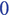°. This is easily accomplished by simply clicking on the value of 0e in the planewave.out file and changing its value from° to°.

Alternatively, the previous changes to the guesses and the reset of pressure phase (0e) to zero could have been accomplished by going directly to the expanded version of the BEGIN segment (#0) and double-clicking on°, which will bring up a “Parameter Edit: 0e Ph(p)” dialog box. Typing “0” into the value and unchecking “Set as Guess” will have the same effect as the procedure using the “Guesses Targets” window. Double-clicking on the frequency will again bring up the “Parameter Edit: 0b Freq” dialog box, and clicking on “Set as Guess” will make (0b) the second “Guess.”

Now run the program again. This time the Run Monitor tells us that DeltaEC made 10 runs in 10 msec. The frequency (0b) has changed to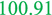Hz (just a bit lower than our estimate of 101.92 Hz, because our half-wavelength calculation ignored dissipation), and |p| has increased to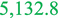Pa from 3853.2 Pa, which was its value when we were near resonance at 100 Hz, but not at resonance.

To demonstrate the versatility of the DeltaEC solver, let’s change the frequency back to 100 Hz, and ask DeltaEC to “tune” the length of the resonator to put the fundamental resonance exactly at 100 Hz at the specified value of the mean gas temperature. We return to the “Guesses Targets” window to “Delete” (0b) from the guess vector and make its value 100.0 Hz. This time, click on the value of the duct length (2c). When the Parameter Edit window opens, you will have the option of checking a box which says “Set as Guess.” Check the box. If you look at the “Guesses Targets” window, you will see that the DUCT: Length (2c) is now a guess.

Run the program again to now calculate the length that the DUCT segment would be required to make 100.0 Hz the resonance frequency. As expected, to lower the frequency by just under 1%, the length has grown by just under 1% to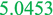m.

In a lossless plane wave resonator with rigid ends and uniform cross-sectional area, the higher resonances corresponding to integer numbers of half-wavelengths fitting between the ends to produce a harmonic series of resonance frequencies, fn = nf1, where n = 1, 2, 3, …∞, just as we observed with the fixed-fixed string in Sect. 3.3.1. To have DeltaEC calculate a few of these overtones, remove DUCT: Length (2c) from the guesses, replace it with BEGIN: Freq (0b) as the “guess,” and then change the value of (0b) in the file to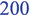Hz. If you run again, DeltaEC finds f2 =Hz. Modify frequency (0b) again to beHz to look for f3 =Hz. The reason the sequence of harmonics is not exactly in integer ratios is that there is dissipation and dispersion within the resonator. We will be able to understand (calculate!) these effects from the acoustic solutions of the hydrodynamic equations once dissipation has been introduced in Chap. 9 and the plane wave solutions to those equations are covered in Chap. 10.

### 6.6 State Variable Plots (∗.sp)

We can convince ourselves that DeltaEC has found the fundamental resonance frequency by using another valuable feature of the software. DeltaEC will display plots of the acoustic variables throughout the model by selecting “Plot SP file” from the “Display” pull-down menu. To improve the resolution of those plots, click on “Edit,” and choose “Options” from the drop-down menu. Increase the number of Runge-Kutta steps in the “Nint” box from 10 to 50 and click “OK.” Now “run” again and select “Plot SP file” from the “Display” drop-down menu. In the “header” shown in Fig. 8.25, uncheck Im[p] and check Im[U] to display the acoustic pressure and acoustic volume velocity for the n = 3 mode of this plane wave resonator. As expected, there are three half-wavelengths with the volume velocity being zero at both ends and the acoustic pressure being maximum at both ends.

Figure 8.25 shows that the real component of pressure, Re[p]; the imaginary component of the volume velocity, Im[U]; and acoustic power flow, Edot, have been selected for plotting. Figure 8.26 shows the plots of those selected variables for both the fundamental (half-wavelength) with f1 = 100 Hz and the second mode (two half-wavelengths) with f2 = 200.8 Hz.

### 6.7 Modifying planewave.out to Create Flask500.out

We will modify planewave.out to represent the Helmholtz resonator of Sect. 8.5.2 by removing the two SURFACE segments, changing the gas from helium to air, changing the dimensions of the DUCT to represent the length and cross-sectional area of the neck of the 500 ml flask in Fig. 8.16, and placing a COMPLIANCE segment between the DUCT and the HARDEND to represent the boiling flask’s 500 ml volume.

Start by clicking on thetitle above the BEGIN statement and changing it to “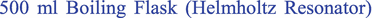” After changing the title, select “Save As” from the “Edit” pull-down menu, and save the file as “500mlFlask.out.”

Under the “Edit” pull-down menu, select “Kill Segment,” then select “1 SURFACE,” and watch it disappear from the file. Now that the first SURFACE is gone, DUCT becomes Segment #1. Double-click on the value of area (1a), change it tom2, and then click “OK.” The neck cross-section is circular, so the perimeter should be Π = 2(πAduct)½ =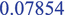m. The Master-Slave link should have done that for you. We will use the physical length of the neck as our DUCT length (1c) =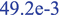m. Finally, click on the title of the segment DUCT and change it to “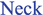” It might be a good idea to save your changes at this point. There is a save icon near the top left of the model.

Some of the variables in the BEGIN Segment #0 also need modification. Again, by clicking on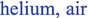can be selected from the menu of gases. Modify Mean P (0a) to be the standard value of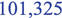Pa and the beginning temperature TBeg (0c) =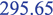°K. Based on the analysis in Sect. 8.5.2, the Helmholtz frequency is expected to be about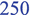Hz, so modify (0b) to reflect that. In the schematic representation of Fig. 8.15, we let the flask be pressure-driven at the open end of the neck. Modify (0d) to be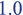Pa,Footnote 17 and uncheck the “Set as Guess” box. Set the magnitude of the volume velocity, |U| at (0f), as a guess, after clearing (0d) from the guess vector. Put |U| “in the correct ballpark” by modifying (0f) to be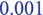m3/sec.

Now all that is left is to “Kill” the other SURFACE that is now in Segment #2, and then insert a COMPLIANCE ahead of HARDEND, which became Segment #2 after the last SURFACE was deleted from the file. Under the “Edit” pull-down menu, select “Insert,” use the dialog box pull-down to select COMPLIANCE, and place it before Segment #2 HARDEND.

Renaming the COMPLIANCE from “” to “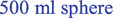” would be an appropriate choice. Since the 500 ml volume is spherical, the surface area is Asphere = $$4\uppi {\left(3V/4\uppi \right)}^{\raisebox{1ex}{2}\!\left/ \!\raisebox{-1ex}{3}\right.}$$ = 3.0465 × 10−2 m2. We should really also subtract the neck area, since that part of the sphere has no surface, so put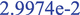m2 in “2a.” The volume is 500 ml =m3. The solid type can remain “.”

Segment #3 can be left as HARDEND, since we do not want any gas to flow out of the end of the volume that is opposite the neck represented by the DUCT in segment #1. Before going further, it would be wise to save this file again. Look over the file to see if you have made any obvious data entry errors (e.g., volume should be 5e-4 and not 5e4), and then hit the “Run” arrow.

### 6.8 Interpreting the ∗.out File

The results of running 500mlFlask.out are shown in Fig. 8.27. In the BEGIN segment (#0), the inputs that were “guesses” have been changed to the values that produced the best agreement with the “targets.” We see that the resonance frequency, defined as the frequency where $$\hat{\mathbf{U}}$$ and $$\hat{\mathbf{p}}$$ were in-phase (specified by 0e = 0 g =°), is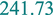Hz. This is close to the result of fo = 245.3 Hz calculated previously using Eq. (8.51).Footnote 18 At resonance, the magnitude of the volume velocity (0f) that enters the neck, driven by a 1.0 Pa (peak) pressure amplitude in front of the neck, is given in (0d) as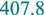cm3/s.

The first four results in the right-hand column of the next three segments will always be the complex pressure and volume velocity at the exit from the segment. At the exit of Segment #1 (where it joins the COMPLIANCE), the magnitude of the pressure is |p| =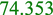Pa. It retains that value throughout the remaining two segments. This is a new result that we could not obtain from our nondissipative analysis of this network in Sect. 8.5.1, and it is important!

In our nondissipative analysis of Eq. (8.52), the ratio of the pressure in the cavity to that in front of the neck diverged at resonance: $$\left|{\hat{\mathbf{p}}}_{cav}/\hat{\mathbf{p}}\right|=\infty$$. Since DeltaEC includes the viscous dissipation caused by the drag of the oscillatory air flow within the neck, and the thermal relaxation losses due to thermal conduction between gases undergoing adiabatic temperature changes, derived in Eq. (7.25), within the volume, the pressure amplitude is now finite. In fact, the “gain” is the quality factor of the Helmholtz resonator, $$Q=\left|{\hat{\mathbf{p}}}_{cav}/\hat{\mathbf{p}}\right|=$$ (2A)/(0d) ≅ 74 (or + 37.4 dB). This pressure increase over a narrow frequency band is just what Helmholtz sought by “plugging” the resonators that share his name into his own ear.

The amplitude of the gas displacement in the neck, ξ1, can be determined by “integrating” the volume velocity divided by the product of the neck area times the angular frequency: $$\left|\hat{\boldsymbol{\upxi}}\right|=\left|\hat{\mathbf{U}}\right|/\left(2\pi {fA}_{neck}\right)=0.547\kern0.5em \mathrm{mm}$$. That is about 1.1% of the total neck length, so our assumption of a 1.0 Pa excitation in (0d) was well within our assumption of linear behavior.9

### 6.9 The RPN Segment

One of the most powerful features of DeltaEC is its ability to perform user-defined calculations within any model using the variable values calculated by the program. Such calculations can be done automatically within the program using the RPN segment available in DeltaEC. The serious student is referred to the DeltaEC User’s Guide for a detailed discussion of the RPN segment, including tables that summarize the wide variety of accessible variables, convenient variable abbreviations for thermophysical properties and state variables (e.g., frequency, mean temperature, power flows, pressure, volume velocity, etc.) included in Table 11.2 of the User’s Manual, and executable mathematical functions (e.g., square roots and other real and complex algebraic operations, circular and hyperbolic trigonometric functions, Bessel functions, logs, and exponentials) also included in Tables 11.3 through 11.7 of the User’s Manual.

To illustrate, an RPN segment has been added to 500mlFlask.out and the file saved as 500mlRPN.out. That RPN segment, shown in Fig. 8.28, automatically calculates the peak-to-peak displacement, $$2\left|\hat{\boldsymbol{\upxi}}\right|$$, of the gas in the resonator’s neck. To eliminate the need for parentheses, the RPN segment uses Reverse Polish Notation:Footnote 19 variables are “declared,” and then an operation on the variable (e.g., taking the cosine of an angle) is executed. If an operation requires two variables (e.g., multiplying one variable by another), then both variables appear before the operation.Footnote 20

For example, the magnitude of the volume velocity entering the neck, $$\left|\hat{\mathbf{U}}\right|$$, that is provided in (0f), must be divided by the cross-sectional area of the neck in (1a), to obtain the average gas velocity in the neck. That velocity then needs to be integrated, so that peak gas velocity must be by divided by ω to obtain the peak gas displacement, $$\left|\hat{\boldsymbol{\upxi}}\right|$$. Since the desired result is the peak-to-peak displacement, $$2\left|\hat{\boldsymbol{\upxi}}\right|$$, the result of the integration must be multiplied by two. In algebraic notation, 2ξ = 2∗(0f)/[w ∗(1a)].Footnote 21 If the result is to be displayed in millimeters, the entire expression must be multiplied by 1000.

In RPN, that same calculation is written, 2ξ = 2(0f)∗(1a)/w/1000 ∗ or 2ξ = 2000 0f ∗ 1a / w /, to provide the result in millimeters. In the first version, the number “2” and (0f) are multiplied (∗), and then (1a) divides (/) the previous result. The RPN abbreviation for ω, which is “w,” divides (/) that result, followed by “1000” and a multiplication (∗). For that RPN segment shown in Fig. 8.28, “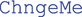” has been replaced by “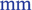,” representing millimeters in the “units” column as a reminder that the result is given in (3A) as millimeters.

That RPN segment (#3) is shown along with the other “collapsed” segments of the model in Fig. 8.28. The RPN segment result (3A) is exactly twice what was calculated for $$\left|\hat{\boldsymbol{\upxi}}\right|$$ “by hand” from the results in the ∗.out file in Fig. 8.27.

### 6.10 Power Flow and Dissipation in the 500 Ml Boiling Flask

The magnitude of the volume velocity, $$\left|\hat{\mathbf{U}}\right|$$, that leaves the neck (1C =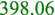cm3/s) is slightly reduced from the value that entered the neck (1f =cm3/s) due to the compliance of the gas in the neck itself. It is worth noticing that in the compliance, $$\hat{\mathbf{U}}$$ and $${\hat{\mathbf{p}}}_{cav}$$ are almost exactly 90° out-of-phase (1B), as they should be for a compliance described in Eq. (8.25). Of course, the volume velocity exiting the compliance is zero ($$\left|\hat{\mathbf{U}}\right|$$ <m3/s) to satisfy the HARDEND condition in Segment #3 of Fig. 8.27 and Segment #4 in Fig. 8.28.

The next two results shown in Fig. 8.27 are Htot (1E) and Edot (1F). Edot is the acoustic (mechanical) power that exits the segment, and Htot is the total power (effectively the sum of the acoustical power plus thermal power converted from acoustical power by dissipation) leaving the segment.Footnote 22 Following Sect. 1.5.4, the acoustic power is one-half of the product of the acoustic pressure magnitude, $$\left|\hat{\mathbf{p}}\right|$$, times the volume velocity magnitude, $$\left|\hat{\mathbf{U}}\right|$$, times the cosine of the phase angle between those quantities. Since $$\hat{\mathbf{U}}$$ and $$\hat{\mathbf{p}}$$ are in-phase, the input acoustical power is $${\left\langle {\Pi}_{in}\right\rangle}_t=\left|\hat{\mathbf{p}}\right|\left|\hat{\mathbf{U}}\right|/2$$ = (0d)∗(0f)/2 = 203.88 μW. Energy is conserved so the total power, Htot, that moves through our resonator is fixed, hence (1E) = (2E) = (3E) =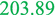μW. That total power cannot exit the model, but at the end of the model has been converted entirely to heat by thermoviscous dissipative processes.

The power dissipated in the neck is the difference between the acoustic power that entered from the BEGIN segment (1E =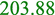μW) and what exited from the neck (1F =μW) and entered the COMPLIANCE. Therefore, those viscous losses in the neck dissipated 173.96 μW, and that power was deposited on the neck as heat and/or swept away and dumped elsewhere by thermoacoustic boundary layer processes , which are beyond the scope of this textbook. Since the walls of the neck are “ideal” and have infinite heat capacity, the temperature of the neck did not increase. Thermoacoustic heat transport can actually cause portions of the duct to cool even in the presence of viscous heating .

The thermal relaxation dissipation in the compliance can be determined by subtracting Edot that leaves the compliance (2F) from Edot that enters (1F):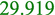μW –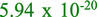W = 29.919 μW. That heat is deposited on the walls of the COMPLIANCE.

Apparently, for this Helmholtz resonator, 85% of the dissipation in the model is due to the viscous losses produced by the oscillatory gas motion in the neck. At this point, radiation losses from the neck of the resonator have not been calculated.Footnote 23

### 6.11 An “Effective Length” Correction

At this point, you should be able to try a few things with DeltaEC on your own (or with the few prompts that follow). Let’s have DeltaEC adjust the length of the neck so that the resonance frequency becomes the measured value, fexp = 213.8 Hz. This can be accomplished by opening 500mlFlask.out, then inserting another DUCT of zero length ahead of the existing DUCT segment representing the neck, and naming that new segment “.” It would also be a good time to rename the title of the model (Line #1) as “.” Use “SaveAs” in the “File” drop-down menu to save the file as “FlaskEffLength.out.”

As a convenience, DeltaEC will add a “Master-Slave Link” to the relationship between area and perimeter to keep the shape of the inserted duct circular. We do not want that link because we do not want the “effective length duct” to add any thermoviscous dissipation. To sever the link, just click onand select “none.”Footnote 24

In the new file, make the new effective length DUCT’s area (1a) be “” by double-clicking on the value of (1a). This will bring up the “Parameter Edit” window for (1a). Click on the “SameAs” button and place “2a” in the window. The “SameAs” feature is very convenient, since various segments of a model whose dimension should be linked can be changed by changing only one variable in one segment. Set the perimeter to an arbitrarily small value, (1b) =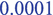m, since the effective length correction is not a “physical” duct that would introduce additional thermoviscous loss.Footnote 25 Change the value of frequency in (0b) to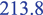Hz, and remove frequency (0b) from the guess vector. Let the length of the “effective length” duct (1c) be a “guess.” Just double-click on the value of (1c) and check the “Set as Guess” box when the “Parameter Edit” window opens. Figure 8.29 shows the ∗.out file produced after that model has run successfully.

The resulting effective length correction, (1c) = 13.54 mm, slightly less than the value (15.5 mm) obtained when we used the expression in Eq. (8.53), which ignored dissipation. Again, less “effective length” was required to obtain the experimentally measured frequency since some of the necessary frequency reduction was provided by gas compliance and viscous dissipation in the neck and thermal-relaxation effects within the 500 ml volume. We will calculate the effective length correction in Sects. 12.8 and 12.9.

### 6.12 Incremental Plotting and the ∗.ip File

Our initial exploration of DeltaEC’s capabilities will conclude by using the software to create a plot of the pressure magnitude and phase within the 500 ml volume as a function of frequency. DeltaEC produces two types of plots: One is the “State Plot,” introduced in Sect. 8.6.6, that allows all of the different results for an individual run to be plotted, usually as a function of position along the apparatus (e.g., real and imaginary pressure magnitude, Edot, etc.). The State Plot is particularly useful for models that are complicated and contain branching and thermoacoustic elements, such as heat exchangers, that change energy flows and temperatures throughout the apparatus being modeled. They also provide essential confirmation of the normal mode shapes for standing waves in complex networks (see Fig. 8.26).

The other plot type is the “Incremental Plot.” An incremental plot lets the user to choose two variables (called the “outer” and “inner” plotting variables) that can be incremented or decremented over a range of equally spaced values. One choice might be a range of static pressures (outer plot variable) that are used to generate plots over a specified range of frequencies (inner plot variable) for each pressure. In the following example, only one (outer) plot variable, the frequency (0b), will be incremented (or decremented) to generate a resonance response curve similar to Fig. 2.12 for the damped simple harmonic oscillator.

The magnitude (2A) and phase (2B) of the pressure inside the compliance of the 500mlFlask.out file in Fig. 8.27 will now be set up to be plotted as a function of frequency (0b). The magnitude of the pressure in front of the neck (0d) will remain constant at 1.0 Pa as the frequency is being swept below and above the resonance frequency. Since the resonance occurs around 240 Hz, the plot will be set up to go from 230 Hz to 250 Hz, placing the Helmholtz resonance frequency roughly in the middle of the plotting range.

It is a good idea to have a model that is converged at the starting point before attempting an incremental plot. Since the plot will start at 230 Hz, click on (0b) and set it to 230 Hz and be sure it is not designated as a “Guess.” The phase could be positive or negative or any phase value that is modulo an integer multiple of 360°, but the phase, though correct, may be inconvenient for display of the plotting results. To avoid phases that exceed +360° or are less than −360°, click on (0 g), and set it to zero degrees. Then run the model to be sure that it converges at the starting point (230 Hz) for the plot. Seeing that the model converged and produced an initial phase between the pressure in front of the neck and the volume velocity at the neck’s entrance of about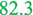°, the model is ready to begin plotting.

To set up the plot, make the magnitude (0f) and phase (0 g) of the volume velocity “guesses.” Then double-click on theHz (0b). In the “Parameter Edit” window, check the “Incr Plot” box. That choice will launch the “Incr(emental) Plot Editor” window. Since the last run of the model was at 230 Hz, DeltaEC will assume that the initial value of the frequency plotting range is 230 Hz, so that frequency will automatically appear in the “From” window. To set the plotting range between 230 Hz and 250 Hz, put 250 Hz in the “To” window, and then set the number of plotting points (#Points) to 81. By specifying 81 points, a step size of 0.25 Hz/point is automatically displayed. An “OPlt” designator will appear to the right of (0b) in the ∗.out file to indicate that frequency is now an independent (outer) plotting variable. Save this new model as 500 mlFlask(Plot).out to distinguish it from previous models.

DeltaEC will automatically include the guesses in the plot file and will tabulate the results in a text file that will be automatically designated 500 mlFlask(Plot).ip. In this case, those guesses are the magnitude (0f) and phase (0 g) of the volume velocity driven through the neck by the externally applied 1.0 Pa pressure. Since we want to plot the magnitude (2A) and phase (2B) of the pressure within the compliance, we click on those results, and check the “Plot(Dependent)” box in the “O(uter)Par(ameter) edit” dialog box. This should produce a “P” to the left of (2A) and (2B) indicating that these results will now also be contained in the incremental plot file.

To check the plotting setup, go to the “Display” pull-down menu, and select “Incremental Plot Sum(mary).” That will produce the Incremental Plot Summary window reproduced in Fig. 8.30.

When you click on the run arrow, DeltaEC will run itself 81 times in about one-half second, ending at 250 Hz, and will have created a new incremental plot file and named it 500mlFlask(Plot).ip. You can examine the content of this plot file in a variety of ways. It can be “opened” in your favorite commercial plotting program (e.g., Excel™) or text editor (e.g., WordPad or NotePad), or it can be examined within DeltaEC using the native DeltaEC plotting software by clicking on “Display” and selecting the “Plot IP file” from the pull-down menu. Figure 8.31 shows a portion of the ∗.ip file, opened with a text editor, containing the first 13 results between 230 and 233 Hz.

If you repeat the plot or if you have plotted the file previously, DeltaEC will provide the option of “overwriting” the existing ∗.ip file, or you can choose to “append” this new run to the previous file (a useful option if you are spanning a large frequency range that you have broken up into shorter runs). If you want to keep the original file, DeltaEC will provide the option to “Rename” the new file.

Checking the file using DeltaEC’s built-in plotter is convenient, since it is a faster way to examine the plot than to export the file to another spreadsheet or mathematics software package. I always check the plotted data with DeltaEC’s indigenous plotting function to make sure that I have plotted the data that I wanted over my range of interest. A DeltaEC-generated incremental plot from the example just run is shown in Fig. 8.32. That incremental plotting window lists the plot variables that can be selected for plotting by checking the desired boxes. In this example, BEGIN:Freq@0b has been selected as the x axis for the plot. COMPL|p|@2A and COMP:Ph(p)@2B are chosen as the y axis variables.

The “windows” below the variables provide a variety of options for scaling the plot. In all three cases, the variable values in Fig. 8.32 are not scaled, hence the “_” symbol in that window. The window below the y axis variables allows selection of the shape, the size, and the color of the plotted points, as well as the options for a line to connect the points. The line color and style (e.g., solid, dashed, dotted, dash-dot) and line width can also be selected by the user. Under the “Options” drop-down menu, “Enable Legend” has been selected to produce the legend in the upper-right corner of the plot in Fig. 8.32. The legend identifies the plotted dependent variables as well as their units and scaling.

Since DeltaEC automatically includes thermoviscous dissipation, the 180° phase shift from below to above the resonance frequency is no longer discontinuous as predicted by the nondissipative result in Eq. (8.52). As shown in Fig. 8.32, the phase changes smoothly through resonance with the largest rate of change of phase as a function of frequency occurring at the resonance frequency. This is demonstrated in Fig. 8.33, where the phase of the plotted frequency closest to the resonance (241.75 Hz) and the phases of the two adjacent frequencies calculated in 500mlFlask(Plot).ip are fit to a straight line.

Agreement between the quality factor based on the amplitude gain and the quality factor based on the slope of the phase vs. frequency would be improved if smaller frequency increments around resonance were selected in the DeltaEC plotting file.

### 6.13 So Much More Utility in DeltaEC

DeltaEC is a very versatile and powerful (and free!) computational tool that is provided with extensive documentation. This introduction could not really demonstrate the full power of the software, but it should have provided the minimum background for its further exploitation in this textbook and in your careers as acousticians. Students are encouraged to download and print parts of DeltaEC User’s Guide, such as the Reference Section, which describes the use of the various segments and the section on the RPN Segment (User’s Guide Chap. 4).

The RPN segment permitted the user great flexibility in calculating quantities of interest automatically within a DeltaEC model every time it is run. It also produces potential targets (e.g., phase differences) that might be more appropriate targets for the solver to use in making the model conform more closely to the behavior of the physical apparatus.

The next two sections will use DeltaEC to analyze two more “lumped-element” networks. They were chosen because they employ inertances and compliances and because they would be rather tedious to analyze without the assistance of DeltaEC.

## 7 Coupled Helmholtz Resonators

The Helmholtz resonator is the fluid analogy of the mass-spring simple harmonic oscillator. The mass of the gas in the neck (see Sect. 8.4.4) is acted upon by the gas in the volume which provides a restoring force as a gas spring (see Sect. 8.2.4). After treating single degree-of-freedom harmonic oscillators in Chap. 2, we went on to analyze coupled oscillators with j masses connected to j + 1 springs in Sect. 2.6. We can do the same with coupled Helmholtz resonators. A simple physical example, built by Anthony Atchley, that has three necks (masses) and four volumes (gas springs) is shown in Fig. 8.34.

To obtain an approximate idea of what frequencies to expect, we can analyze a double-Helmholtz resonator that is created when one neck is connected to two identical volumes [22, 23]. That network is equivalent to a single (gas) mass restored by two mechanically parallel (gas) springs (see Sect. 2.2.1). Since two springs of equal stiffness provide a stiffness that is twice that of each individual spring, Eq. (8.51) can be modified to calculate the resonance frequency, ωDouble.

$${\omega}_{Double}=\frac{1}{\sqrt{LC}}=c\sqrt{\frac{2A}{\Delta {x}_{neck}V}}$$
(8.55)

Using parameter values taken from the caption below Fig. 8.34, the volume of a single compliance is V = 2.04 × 10−5 m3. The neck has a length, Δxneck = 19 mm, and cross-sectional area, A = 4.6 × 10−5 m2. If the air temperature is 23 °C, then c = 345 m/s, and fDouble = ωDouble/2π = 846 Hz.

With three necks and four gas springs, the triple-Helmholtz resonator has three degrees of freedom and therefore will possess three lumped-element normal mode frequencies. The DeltaEC model, shown in “Schematic View” in Fig. 8.34, can be run to determine the three resonance frequencies, and three ∗.sp. files (see Sect. 8.6.6) can be generated showing the gas’s volume velocity magnitudes corresponding to the three normal modes. Those normal modes are shown in Fig. 8.35, along with the analogous displacements of three discrete masses connected together by strings.

Although the second normal mode is similar to two double-Helmholtz resonators, oscillating 180° out-of-phase, with the gas in the central neck at rest, examination of the ∗.sp. file for that mode, in Fig. 8.36, shows that in such a small network, with a high ratio of surface area to volume, the thermoviscous losses are significant. The previous lossless analysis of the double-Helmholtz resonator shows that in such a network, the normal mode frequency should be 846 Hz. The DeltaEC model places that normal mode resonance frequency at 671 Hz. It is clear from this state variable plot in Fig. 8.36 that the gas in the central neck is not at rest and the pressure on opposite ends of the left pair of volumes, which would be equal and opposite for the lossless case, is unequal in magnitude (28.8 Pa vs. 22.5 Pa). The same is true for the right pair of volumes (21.0 Pa vs. 18.2 Pa).

## 8 The Bass-Reflex Loudspeaker Enclosure

As will be shown in greater detail later (see Sect. 12.5.1), a moving-coil electrodynamic loudspeaker is a very inefficient source of sound at low frequencies if it is not surrounded by a rigid enclosure. Such an enclosure allows only the front surface of the speaker’s cone to radiate into the listening space and suppresses the out-of-phase volume velocity produced by the rear of the speaker that would otherwise have cancelled the volume velocity created by the front of the speaker. This strategy is illustrated on the left-hand side of Fig. 8.37. One unfortunate consequence of such strategies is that the volume velocity produced by the back of the loudspeaker, though just as large as that produced by the front, is “wasted.”

The phase reversal produced when a Helmholtz resonator is driven above its resonance frequency, ωo, shown in Eq. (8.52) and plotted in Fig. 8.32, can productively utilize the volume velocity produced by the back of the loudspeaker. Such a bass-reflex loudspeaker enclosure, shown on the right-hand side of Fig. 8.37, exploits this phase reversal by taking the volume velocity generated from the rear of the loudspeaker’s cone and inverting its phase, so the motion of the gas oscillating in the port (i.e., the neck of the Helmholtz resonator) adds (nearly in-phase) to the gas being driven by the front of the loudspeaker cone. At low frequencies, the separation of the cone’s center and the vent is much less than one-half wavelength, so the volume velocity exiting the port will combine (using vector algebra to incorporate the phase differences) with the volume velocity produced by the front of the speaker to produce the net volume velocity magnitude, |Unet|, that can exceed the volume velocity produced by the front of the loudspeaker.

There are several other technical issues that need to be considered for successful design of a bass-reflex loudspeaker enclosure that will not be addressed here. For example, the free-cone resonance of the speaker is strongly coupled to the Helmholtz resonance, and inclusion of damping material in the port can be useful in smoothing the overall response. (DeltaEC will automatically incorporate those effects for us if we specify the flow resistance of the damping material in the port as shown in Segment #3 of Fig. 8.39.) Since the volume velocity through the port can be substantial at frequencies close to the (strongly coupled) Helmholtz resonance frequency, flow noise generated by turbulence in the port and jetting caused by the high-speed gas flow in the port can be annoying. That flow noise is referred to by audio component manufacturers as the “port noise complaint” .

### 8.1 Beranek’s Box Driven by a Constant Volume Velocity

It will be worthwhile to pursue this application a little further because it is an example of a Helmholtz resonator that is driven in a way that is different from the external pressure drive of our first example, shown schematically in Fig. 8.15 and modeled by DeltaEC in Figs. 8.27 and 8.29. A schematic diagram of a Helmholtz resonator being driven by a volume velocity source feeding the interior of the compliance is shown in Fig. 8.38. This configuration places the compliance of the volume and the inertance of the port acoustically in parallel. The volume velocity, |U |, provided by the rear of the speaker cone, goes simultaneously toward compressing the gas in the volume and driving gas through the neck (port).

To illustrate a Helmholtz resonator driven by a volume velocity source located within the compliance, a crude DeltaEC model of the bass-reflex enclosure is developed to represent the example in Beranek’s Acoustics textbook . It’s crude because it assumes that the loudspeaker produces a constant volume velocity of 0.040 m3/s, which is independent of frequency. In fact, the volume velocity of the loudspeaker is frequency-dependent, due to the mass, stiffness, and damping (mechanical impedance) of the loudspeaker (see Sect. 2.5.5). The frequency dependence of the complex input electrical impedance of the loudspeaker’s voice coil and associated magnet structure also modifies the current, hence the resulting force, if the coil is driven by a source of constant voltage. These effects will be ignored initially to demonstrate the phase-inversion effect of the bass-reflex approach. In the next section, a real loudspeaker (JBL 2242 PHL, S/N: J033N-51645), easily modeled using DeltaEC, will be used to excite the same enclosure in a more realistic way from a “constant voltage” source.

This example will also ignore any damping material (e.g., fiberglass) that might be used to line the interior surface of the compliance. Such material is designated in Fig. 8.37 (Right) as “acoustic absorber.” That material is used to suppress standing waves within the enclosure (see Sect. 13.1), which occur at frequencies much higher than those which we consider here for the bass-reflex enclosure’s behavior.

Beranek’s speaker example has an effective piston area, Acone = 8.03 × 10−2 m2, and his enclosure has an internal volume, V = 0.31 m3 (30″ × 35″ × 18″). The surface area of this acoustical compliance is 2.86 m2. (This surface area could be increased in a DeltaEC model to represent the “acoustic absorber” that is included to suppress standing waves within the enclosure that occur at frequencies that are much higher than those of interest in the analysis of the bass-reflex behavior.) Beranek’s port area, Sp = 0.055 m2, corresponding to a port diameter of about 8 cm (3.14″). The port has a length of 0.25 m (9.8″), neglecting any end corrections. An acoustic flow resistance of 500 Pa-s/m3 (see Segment #3) has been added to the port to control the behavior at resonance.Footnote 26 These parameter choices are reflected by the output file, BeranekBox(U-drive).out, shown in Fig. 8.39.

Care must be taken to understand the phase differences between the volume velocity of the source (set at 0° in BEGIN), $${\hat{\mathbf{U}}}_{\mathbf{drive}}$$, and the volume velocity produced by the front of the loudspeaker. The front of the loudspeaker is moving in the direction opposite that of the back side that produces $${\hat{\mathbf{U}}}_{\mathbf{drive}}$$. The net volume velocity, Unet, must be the vector sum of the volume velocity from the front of the loudspeaker plus the volume velocity of the gas moving through the port. The magnitude of the net velocity can be calculated using the Law of Cosines.

$${c}^2={a}^2+{b}^2-2 ab\cos \phi \kern1em \Rightarrow \kern1em {U}_{net}^2={U}_{drive}^2+{U}_{port}^2-2\left|{U}_{drive}\right|\left|{U}_{port}\right|\cos \phi$$
(8.56)

To calculate the phase of the volume velocity through the port, Uport, relative to the volume velocity from the front of the loudspeaker, Uspeaker = −Udrive, it is helpful to recognize that Udrive and Uport will be in-phase at frequencies well below ωo. Therefore, the phase difference between Uspeaker and Uport at any frequency, ϕ(f) = ϕdrive + 180° – ϕport = 180° – ϕport, since ϕdrive = 0°, by definition in the BEGIN segment (0 g). The full calculation is executed by DeltaEC from the file BeranekBox(U-source).out, shown in Fig. 8.39. The results of those calculations are plotted in Fig. 8.40.

The enhancement of the bass response, shown by the fact that net volume velocity exceeds the volume velocity from the front of the speaker’s cone at frequencies above about 35 Hz, helps compensate for the decrease in the sensitivity of human hearing at low frequencies (see Fig. 10.5).

### 8.2 Loudspeaker-Driven Bass-Reflex Enclosure*

It will be worthwhile to place a real loudspeaker in Beranek’s bass-reflex loudspeaker enclosure as the last example in this chapter. Techniques for measurement of electrodynamic loudspeaker parameters were demonstrated in Sect. 2.5.5. DeltaEC provides a selection of segments that easily incorporate a loudspeaker into a DeltaEC model. The resulting combination of an electromechanical harmonic oscillator and a fluidic Helmholtz resonator presents challenges if approached algebraically. We will see that incorporation of a loudspeaker in a Helmholtz resonator within DeltaEC is no more difficulty than the model of Beranek’s bass-reflex enclosure that was run with a constant amplitude volume velocity source in the BEGIN statement of the model shown in Fig. 8.39. Although the use of such a DeltaEC model for optimization of the system’s performance can be more complicated, just “plugging in” the appropriate DeltaEC segment to represent the loudspeaker is simple.

In this example, the measured parameters of a JBL Model 2242 PHL (S/N: J033N-51645) will characterize the loudspeaker in the VSPEAKER segment that is included in BarenekBox-VSpeaker.out, shown in Fig. 8.41. Because modern solid-state audio amplifiers produce a nearly constant voltage replica of the audio signal (at least until the current limit is exceeded), a VSPEAKER segment is used to represent the speaker and amplifier combination. As shown in Fig. 8.41, the loudspeaker is specified entirely by the parametric inputs to Segment #1.Footnote 27 The user must provide the radiating area of the speaker (1a), the DC resistance of the voice coil (1b), the inductance of the voice coil (1c), and the force factor, also known as the Bℓ-product (1d). The moving mass of the cone plus voice coil plus suspension surround and spider (1e) is also required, along with the suspension stiffness (1f) and the mechanical resistance (1g), as well as the amplitude of the driving voltage (1h). Of course, all of the input parameters must be provided to DeltaEC in SI units.

Figure 8.42 provides a graph that includes the speaker’s input electrical impedance (2A) and the magnitude of the volume velocity produced by the front side of the speaker’s cone (1C) when driven by an input voltage of 7.07 Vrms applied to the voice coil that is independent of frequency. The net volume velocity, |Unet|, is produced by the vector sum of the speaker’s cone and the gas oscillating within the enclosure’s port.

The loudspeaker’s mechanical (free-cone) resonance frequency, $${f}_s=\sqrt{\mathrm{K}/m}/2\pi \cong 36.6\kern0.5em Hz$$, if it were measured in a vacuum. The Helmholtz resonance of the enclosure without the loudspeaker (fo = 43.6 Hz) can be determined from BeranekBox(U-source).out in Fig. 8.39. A first approximation to a bass-reflex loudspeaker enclosure design usually makes the Helmholtz frequency of the enclosure (with the loudspeaker immobilized) roughly equal to the speaker’s free-cone resonance frequency. No attempt was made to “tune” the enclosure, possibly by modifying the port’s dimensions or its damping, to enhance the loudspeaker’s performance, but it is obvious from inspection of Fig. 8.42 that above 33 Hz, the net volume velocity produced by the speaker/enclosure combination is greater than that produced by the front radiating surface of the loudspeaker only, without any (conscious) optimization effort.

The dash-dotted curve in Fig. 8.42, representing the magnitude of the input electrical impedance, |Zin|, of the loudspeaker’s voice coil, shows two peaks corresponding to the two normal mode frequencies of a two degree-of-freedom coupled harmonic oscillator (see Sect. 2.7). The two independent resonance frequencies of the Helmholtz resonator alone, fo, and the free-cone resonance frequency of loudspeaker alone, fs, differ by Δf independent = |fofs| = |43.6–36.6| Hz = 7.0 Hz. The separation of the two peaks in the electrical impedance of the loudspeaker, Δfcoupled = (62–27) Hz = 35 Hz. This is a clear manifestation of the “level repulsion” exhibited by two strongly coupled harmonic oscillators that was discussed in Sect. 2.7.6.

The peak in the magnitude of the volume velocity through the port, which occurs at about 45 Hz, corresponds to the dip in the volume velocity provided by the front surface of the loudspeaker, demonstrating that the energy dissipated in the port produces a perceptible additional load on the loudspeaker’s motor mechanism. This loading, of course, was not evident in the DeltaEC model of Fig. 8.39, which assumed a constant value for the driver’s volume velocity.

## 9 Lumped Elements

This (rather long) chapter was intended to accomplish two major goals: First, it provided the initial application of the equations of hydrodynamics to acoustical problems of interest by linearizing the continuity equation and linearizing the Euler equation to produce the acoustical compliance and acoustical inertance of small acoustical elements. The decision to define acoustical impedance as the ratio of the acoustic pressure to volume velocity facilitated the combination of inertances and compliances, since volume velocity is continuous across the junction between lumped elements that typically can have different cross-sectional areas. Though it is true that these elements were small compared to the acoustic wavelength, at the frequencies of interest, as was demonstrated at the end of Chap. 2, combinations of many such elements provide a logical transition to wave motion in distributed systems with dimensions comparable to (or greater than) the wavelength of sound (see Fig. 10.1). Of course, the lumped elements have significant utility within their own domain of applicability.

Second, this chapter also introduced DeltaEC software that could be used to predict the behavior of network of such “lumped elements,” focusing first on the combination of an inertance and a compliance to produce a Helmholtz resonator, driven by external oscillating pressure or by an internal source of volume velocity. DeltaEC provided a computational structure that could be applied to networks of lumped elements and included the effects of thermoviscous dissipation on the surfaces of those elements. Application of DeltaEC to a 500 ml boiling flask provided a “benchmark” problem that we will be able to use to test the hydrodynamic models for dissipative process that will be the subject of the next chapter.

The comparison between our nondissipative model, which produced an expression for a Helmholtz resonator’s resonance frequency, and some simple (but sufficiently accurate) measurements of that frequency exposed a substantial discrepancy between theory and experiment. That discrepancy was removed by postulating an “effective length correction,” since the dependence of the frequency on the volume of the resonator seemed to follow the behavior dictated by the simple nondissipative network calculation. Only “hand-waving” plausibility arguments, appealing to effects of flow adjacent to the resonator’s neck, were provided as “justification.” That is not science! We will need to create a legitimate theory that produces a quantitative “end correction” that can be related to the neck’s radius and the specific geometrical constraints on the flow of fluid into and out of the neck in the vicinity of the neck’s openings. Such a theory will be forthcoming when the radiation from circular pistons is developed in Chap. 12.

### Talk like an Acoustician

 Lumped element Fluid particle or fluid parcel Acoustical compliance Lagrangian description Acoustical inertance Eulerian volume Helmholtz resonator Nonlinear effect Harmonic analysis Streaming Isotropic fluid Adiabatic sound speed Mean value Acoustical impedance Instantaneous value Capacitive reactance Acoustic approximation Atmospheric lapse rate Acoustic Mach number Inductive reactance Fourier’s theorem Acoustical network Laboratory frame of reference Joining conditions Eulerian coordinate system Bass-reflex loudspeaker enclosure

### Exercises

1. 1.

Atmospheric lapse rate. Commercial jet aircraft typically cruise at altitudes around 36,000 feet.

1. (a)

Temperature. What would be the temperature of air at that altitude if we assume a dry adiabatic gas and a sea-level pressure of 100 kPa and sea-level temperature of 15 °C?

2. (b)

Density. Assuming an isothermal atmosphere with a temperature that is the average of 15 °C and the temperature calculated in part (a), what would be the density and pressure of the air at cruising altitude?

2. 2.

Bobbing Hydrophone. Suppose a hydrophone is suspended some distance below a buoy that is floating at the surface of a body of water as shown in Fig. 8.43. If there are waves on the surface that cause the buoy to move in the vertical direction by an amount, $$z(t)=\mathit{\Re e}\left[\hat{\mathbf{A}}{e}^{j\omega t}\right]$$, where $$\left|\hat{\mathbf{A}}\right|=0.20\kern0.5em m$$, determine the amplitude of the pressure signal detected by the hydrophone at the same frequency, ω, if we assume that the separation between the hydrophone and the buoy is constant and the water is incompressible.

3. 3.

Up, up, and away. A thin rigid spherical shell that is 1.00 m in diameter has a mass of 0.50 kg when evacuated. At “sea level” (h = 0) on the surface of the Earth, under typical atmospheric conditions (T = 15.0 °C, P = 101,325 Pa), such a sphere would displace 0.641 kg of air; therefore it is buoyant and would rise. If we assume conditions specified by the 1976 Standard US Atmosphere , then the density of air, ρ(h), would decay exponentially, as described in Eq. (8.31), as a function of height, h, above the Earth’s surface. The density of air at sea level is ρo = 1.225 Kg/m3. The characteristic exponential decay length, μ ≡ ℜT/gM = 8435 m.

1. (a)

Altitude. What is the equilibrium height above the Earth, ho, to which the hollow sphere will rise?

2. (b)

Väisälä-Brunt frequency. Since the equilibrium is stable, if the sphere is displaced from its equilibrium position, its height will oscillate about equilibrium. The effective mass of the oscillating sphere will include a contribution from the motion of the surrounding air. That additional “hydrodynamic mass” is equal to one-half of the mass of air that the sphere displaces (see Sect. 12.5.1) . Assuming negligible damping, what is the period of free oscillation of the sphere when it is displaced (vertically) from its equilibrium position and released if the additional “hydrodynamic mass” of the surrounding fluid is added to the mass of the sphere? The acceleration due to gravity at the equilibrium position can be taken as 9.8 m/s2.

3. (c)

Damping. The drag force on a sphere in a viscous fluid is given (at low Reynolds numbers) by Fvis = 6πηrv, where r is the radius of the sphere, v is its velocity, and η = 1.72 × 10−5 N-sec/m2 is the viscosity of air at the equilibrium height. Determine the decay time, τ, for the oscillations of the sphere to decay to 1/e of their initial amplitude.

4. (d)

Spherical shell strength. The spherical shell is not really rigid. It is made of a carbon fiber composite with density, ρ = 1.6 gm/cm3; Young’s modulus, E = 70 GPa; Poisson’s ratio, ν = 0.1; and ultimate compressive strength of 500 MPa. Can such a shell survive at sea level without imploding? If so, what change in radius occurs as it goes from sea-level pressure to the pressure calculated at the equilibrium height calculated in part (a)?

4. 4.

Pistonphone microphone calibrator. A pistonphone is a handheld instrument commonly used to calibrate microphone systems. As shown in Fig. 8.44, it is essentially a rigid-walled cavity driven at a single frequency by two horizontally opposed pistons that ride on a rotating cam so that each piston has known displacement amplitude. The dimensions of the cavity are all much smaller than the wavelength of sound at the operating frequency. For the purpose of this problem, assume the cavity is cylindrical with a height of 1.50 cm and a radius of 1.80 cm. Let the volume of the cavity be 3.8 cm3, and ignore the space taken by the piston-cam system. Assume the mean pressure in the cavity, pm = 101 kPa, cair = 343 m/sec, and that γair = 1.403.

1. (a)

Piston volume velocity. Treating the cavity as a lossless compliance, what must be the magnitude of the peak (not effective) volume velocity produced by the pistons for the peak sound pressure within the cavity to be 44.8 Papeak (equivalent to a sound pressure level of 124 dBSPL re: 20 μParms) when driven at 250 Hz?

2. (b)

Phase. What is the approximate (to within ±5°) phase difference between the pressure in the cavity and the volume velocity of the piston?

3. (c)

Piston motion. What is the peak-to-peak displacement of each piston if there are two pistons (as shown in Fig. 8.44) and each piston has a diameter of 3 mm, assuming that both pistons have the same displacement amplitudes?

5. 5.

1.0 liter flask. In Sect. 8.5.2, we used Eq. (8.54) to determine the empty resonator volume, Vo, and the effective neck length, Δxeff, by adding known amounts of water to a flask and measuring the corresponding resonance frequency using a microphone inside the flask. [Note: The mic volume was 6 cm3.] Now you can enjoy doing this on your own for this larger flask with the data provided in Table 8.1. The diameter of the neck is 33.85 mm and its physical length is 85 mm.

1. (a)

Effective length. Calculate Vo and Δxeff.

2. (b)

Effective length correction. Calculate the additional length that had to be added to the physical length to produce Δxeff, and also express this length in terms of the radius of the neck.

3. (c)

Water compressibility. Is the compressibility of the water in the flask negligible in comparison to the compressibility of the air? Assume the flask contains 400 mL of water for your calculations.

6. 6.

The Penn State commemorative bottle. The bottle shown in Fig. 8.45 can be modeled in DeltaEC with a DUCT as a neck, a CONE as the transition between the neck and the volume, and another DUCT that has the volume of the end of the bottle closed with a SURFACE segment followed by a HARDEND segment.

1. (a)

Helmholtz resonance frequency. Determine the resonance frequency of the resonator in Fig. 8.45 if the bottle is filled with air at 101,325 Pa at a temperature of 20 °C.

2. (b)

Quality factor. Use the results of the DeltaEC model to find the Q of the Helmholtz resonance (neglecting radiation losses).

3. (c)

Standing waves. Use your DeltaEC model to calculate the frequencies of the three lowest-frequency standing wave resonances of the bottle.

4. (d)

∗.sp plots. Plot the cross-sectional area, GasA; the in-phase pressure magnitude, ℜe [p]; and the out-of-phase volume velocity, ℑm [U], for the Helmholtz mode and the two lowest-frequency standing wave modes.

7. 7.

Double-Helmholtz resonator. The double-Helmholtz resonator shown in Fig. 8.46 was used as a thermoacoustic refrigerator .

1. (a)

Density. The resonator is pressurized with 88% helium and 12% xenon at 3.0 MPa and is at a temperature of 20 °C. Calculate the mean atomic weight of the noble gas mixture, Mmix = x MXe + (1 − x) MHe, were x is the xenon concentration. Use the mean atomic weight to determine the mixture’s density. [Note: You must provide your calculation, but you are welcome to check your answer using the DeltaEC ThermoPhysical Properties.]

2. (b)

Resonance frequency. As a double-Helmholtz resonator with unequal volume compliances, calculate the resonance frequency of the resonator in Fig. 8.46 that is filled with an 88/12 mixture of helium and xenon at 3.0 MPa.

3. (c)

Gas velocity. In this resonator, the pressure ratio, $$\left|\hat{\mathbf{p}}\right|/{p}_m$$, in the right-hand volume was 5.5%. What is the amplitude of the particle velocity of the gas in the neck? Also report that velocity amplitude in miles per hour and as a percentage of the sound speed.

4. (d)

DeltaEC model. Make a DeltaEC model of this double-Helmholtz resonator that is driven by a piston in the left-hand volume with volume velocity 0.010 m3/sec. The surface areas of the compliances are Aleft = 2.3 m2 and Aright = 1.6 m2. [Note: The imposed volume velocity will not produce the full 6 kW mentioned in the caption to Fig. 8.46.]

8. 8.

Helmholtz resonator. In Sect. 8.5.2, the effective length correction for the neck of a Helmholtz resonator and the volume of the resonator were determined by measured variations in the resonator’s volume, a procedure that you were asked to repeat in Problem 5. In this problem, you will do the same, but by substitution of differing necks with carefully measured physical lengths.

Figure 8.47 shows a Helmholtz resonator that is constructed from plumbing fixtures that include 6″ (nominal) Schedule-40 PVC pipe and a 6″ PVC 90° elbow. One end of the resonator is closed with a ¼″ thick PVC plate, and the base is medium-density fiberboard (MDF). The inside diameter of the pipe (1.5″ nominal) used for the necks is Dneck = 40.8 mm. Assume a room temperature sound speed of 343 m/s.

1. (a)

Enclosure volume and effective length correction. Using the data in Table 8.2, determine the volume of the Helmholtz resonator’s compliance and the effective length correction and their relative uncertainties.

2. (b)

Quality factor. Based on the peak-to-peak values of the free-decay amplitudes given in Table 8.3 for each half-cycle of vibration, determine the Q of the resonance.